Methods for an object of class "jm" for diagnostic functions.

traceplot(object, ...)

# S3 method for jm
traceplot(object,
parm = c("all", "betas", "sigmas", "D", "bs_gammas",
"tau_bs_gammas", "gammas", "alphas"), ...)

ggtraceplot(object, ...)

# S3 method for jm
ggtraceplot(object,
parm = c("all", "betas", "sigmas", "D", "bs_gammas",
"tau_bs_gammas", "gammas", "alphas"),
size = 1, alpha = 0.8,
theme = c('standard', 'catalog', 'metro',
'pastel', 'beach', 'moonlight', 'goo', 'sunset', 'custom'),
grid = FALSE, gridrows = 3, gridcols = 1, custom_theme = NULL, ...)

gelman_diag(object, ...)

# S3 method for jm
gelman_diag(object,
parm = c("all", "betas", "sigmas", "D", "bs_gammas",
"tau_bs_gammas", "gammas", "alphas"), ...)

densplot(object, ...)

# S3 method for jm
densplot(object,
parm = c("all", "betas", "sigmas", "D", "bs_gammas",
"tau_bs_gammas", "gammas", "alphas"), ...)

ggdensityplot(object, ...)

# S3 method for jm
ggdensityplot(object,
parm = c("all", "betas", "sigmas", "D", "bs_gammas",
"tau_bs_gammas", "gammas", "alphas"),
size = 1, alpha = 0.6,
theme = c('standard', 'catalog', 'metro', 'pastel',
'beach', 'moonlight', 'goo', 'sunset', 'custom'),
grid = FALSE, gridrows = 3, gridcols = 1, custom_theme = NULL, ...)

cumuplot(object, ...)

# S3 method for jm
cumuplot(object,
parm = c("all", "betas", "sigmas", "D", "bs_gammas",
"tau_bs_gammas", "gammas", "alphas"), ...)

## Arguments

object

an object inheriting from class "jm".

parm

a character string specifying which parameters of the joint model to plot. Possible options are 'all', 'betas', 'alphas', 'sigmas', 'D', 'bs_gammas', 'tau_bs_gammas', or 'gammas'.

size

the width of the traceplot line in mm. Defaults to 1.

alpha

the opacity level of the traceplot line. Defaults to 0.8.

theme

a character string specifying the color theme to be used. Possible options are 'standard', 'catalog', 'metro', 'pastel', 'beach', 'moonlight', 'goo', or 'sunset'. Note that this option supports fitted objects with three chains. If the object was fitted using a different number of chains then the colors are either automatically chosen, or can be specified by the user via the argument custom_theme.

grid

logical; defaults to FALSE. If TRUE, the plots are returned in grids split over multiple pages. For more details see the documentation for gridExtra::marrangeGrob().

gridrows

number of rows per page for the grid. Only relevant when using grid = TRUE. Defaults to 3.

gridcols

number of columns per page for the grid. Only relevant when using grid = TRUE. Defaults to 1.

custom_theme

A named character vector with elements equal to the number of chains (n_chains). The name of each element should be the number corresponding to the respective chain. Defaults to NULL.

...

further arguments passed to the corresponding function from the coda package.

## Value

traceplot()

Plots the evolution of the estimated parameter vs. iterations in a fitted joint model.

ggtraceplot()

Plots the evolution of the estimated parameter vs. iterations in a fitted joint model using ggplot2.

gelman_diag()

Calculates the potential scale reduction factor for the estimated parameters in a fitted joint model, together with the upper confidence limits.

densplot()

Plots the density estimate for the estimated parameters in a fitted joint model.

ggdensityplot()

Plots the evolution of the estimated parameter vs. iterations in a fitted joint model using ggplot2.

cumuplot()

Plots the evolution of the sample quantiles vs. iterations in a fitted joint model.

## Author

Dimitris Rizopoulos d.rizopoulos@erasmusmc.nl

jm

## Examples

# \donttest{
# linear mixed model fits
fit_lme1 <- lme(log(serBilir) ~ year:sex + age,
random = ~ year | id, data = pbc2)

fit_lme2 <- lme(prothrombin ~ sex,
random = ~ year | id, data = pbc2)

# cox model fit
fit_cox <- coxph(Surv(years, status2) ~ age, data = pbc2.id)

# joint model fit
fit_jm <- jm(fit_cox, list(fit_lme1, fit_lme2), time_var = "year", n_chains = 1L)

# trace plot for the fixed effects in the linear mixed submodels
traceplot(fit_jm, parm = "betas")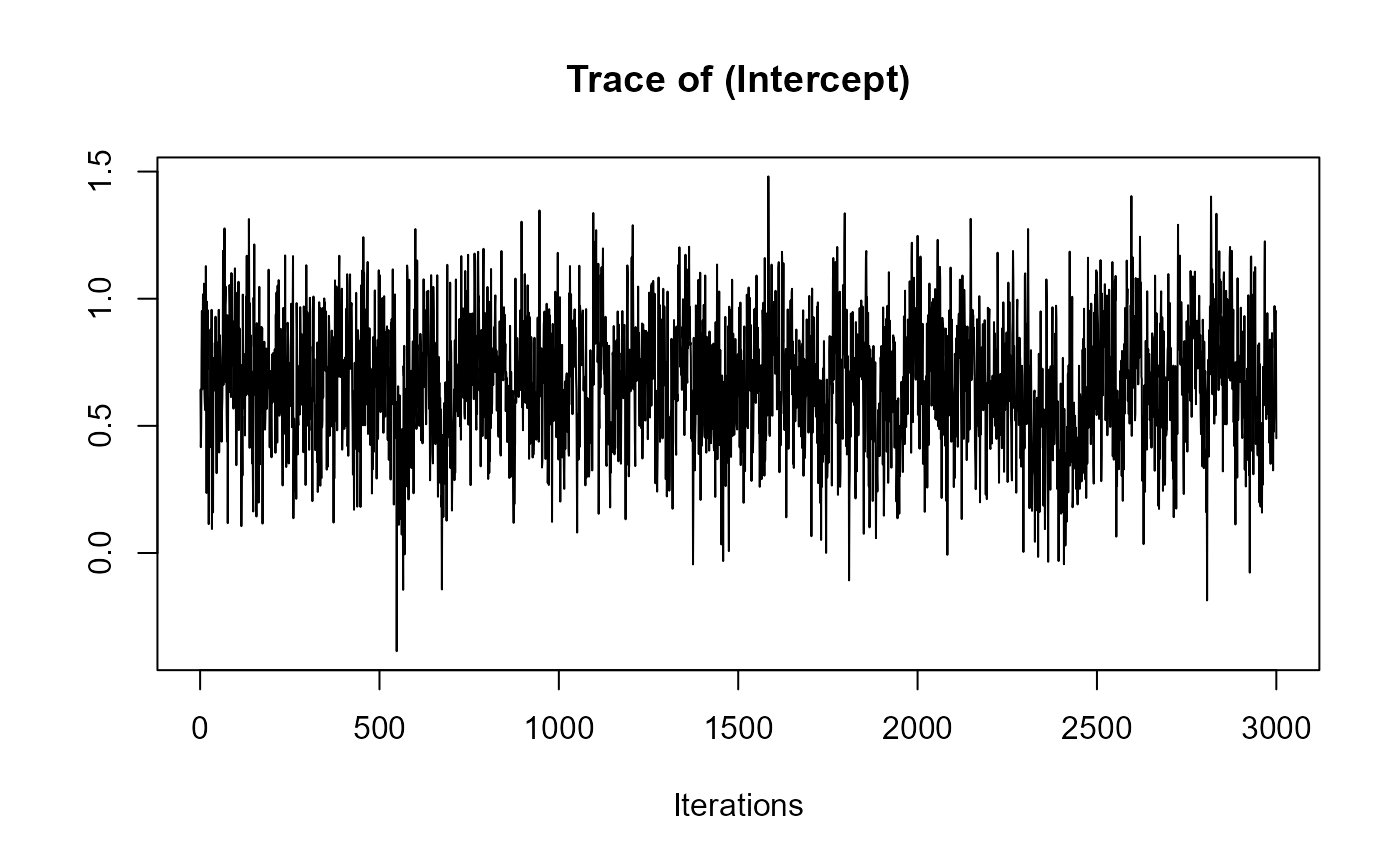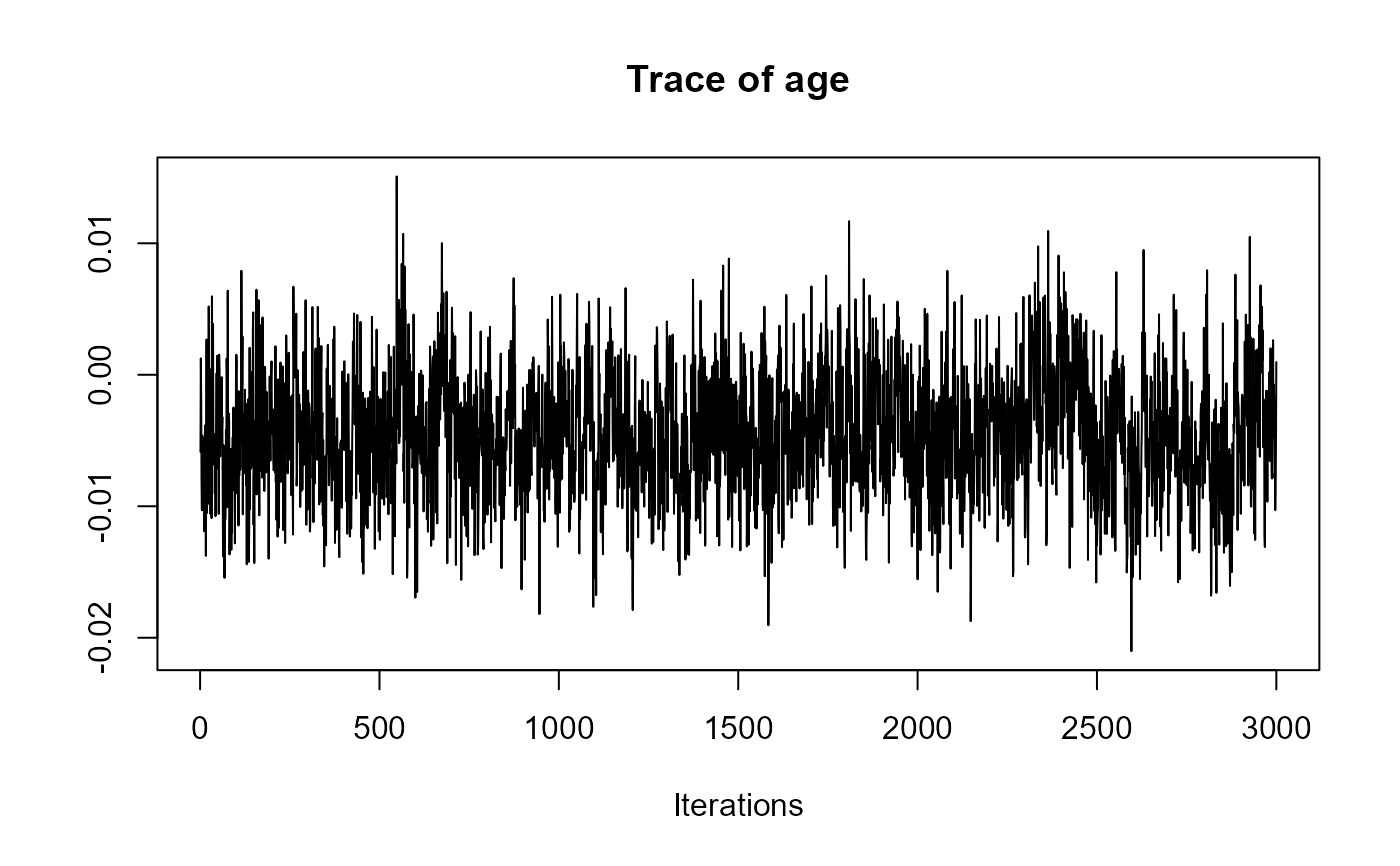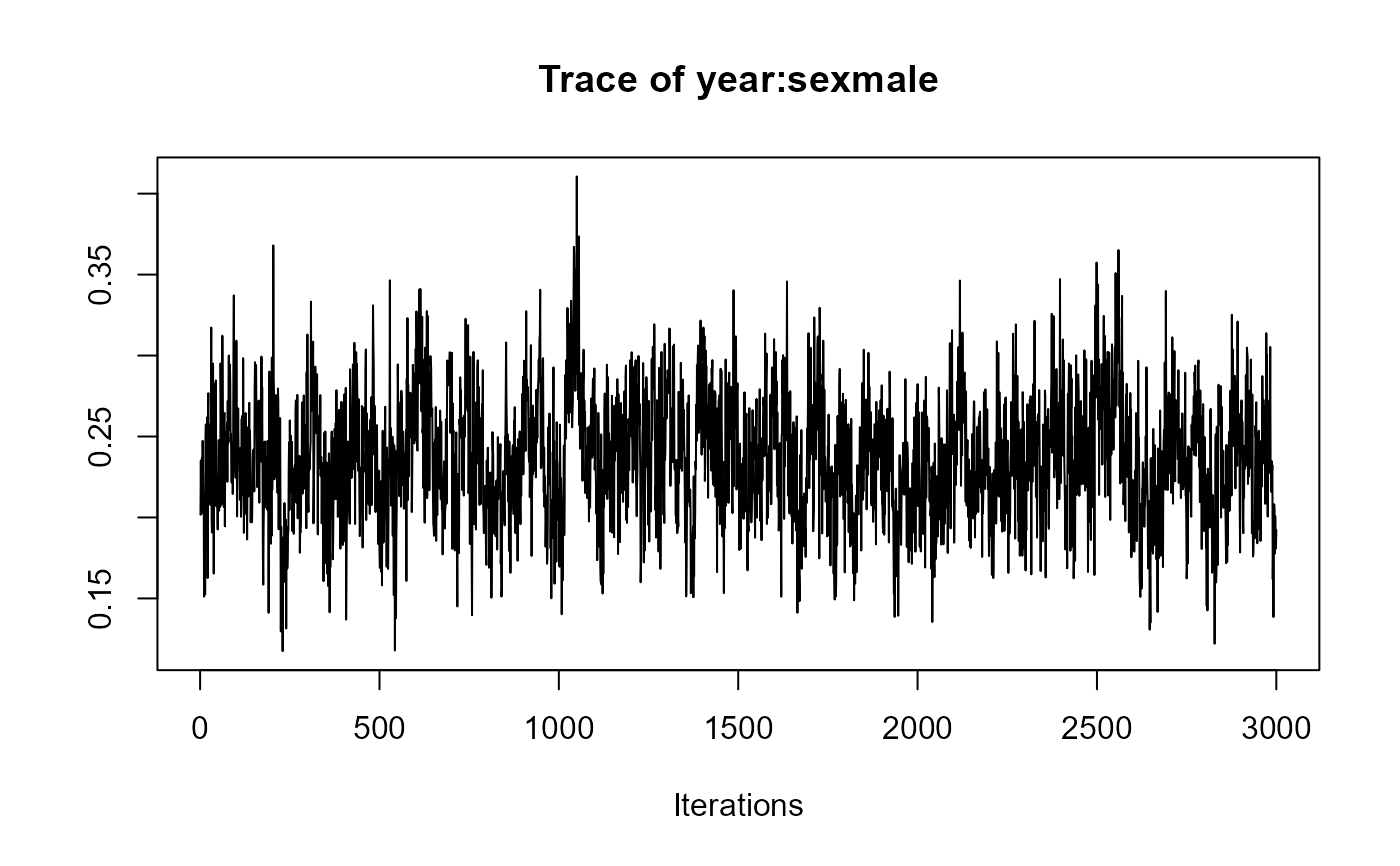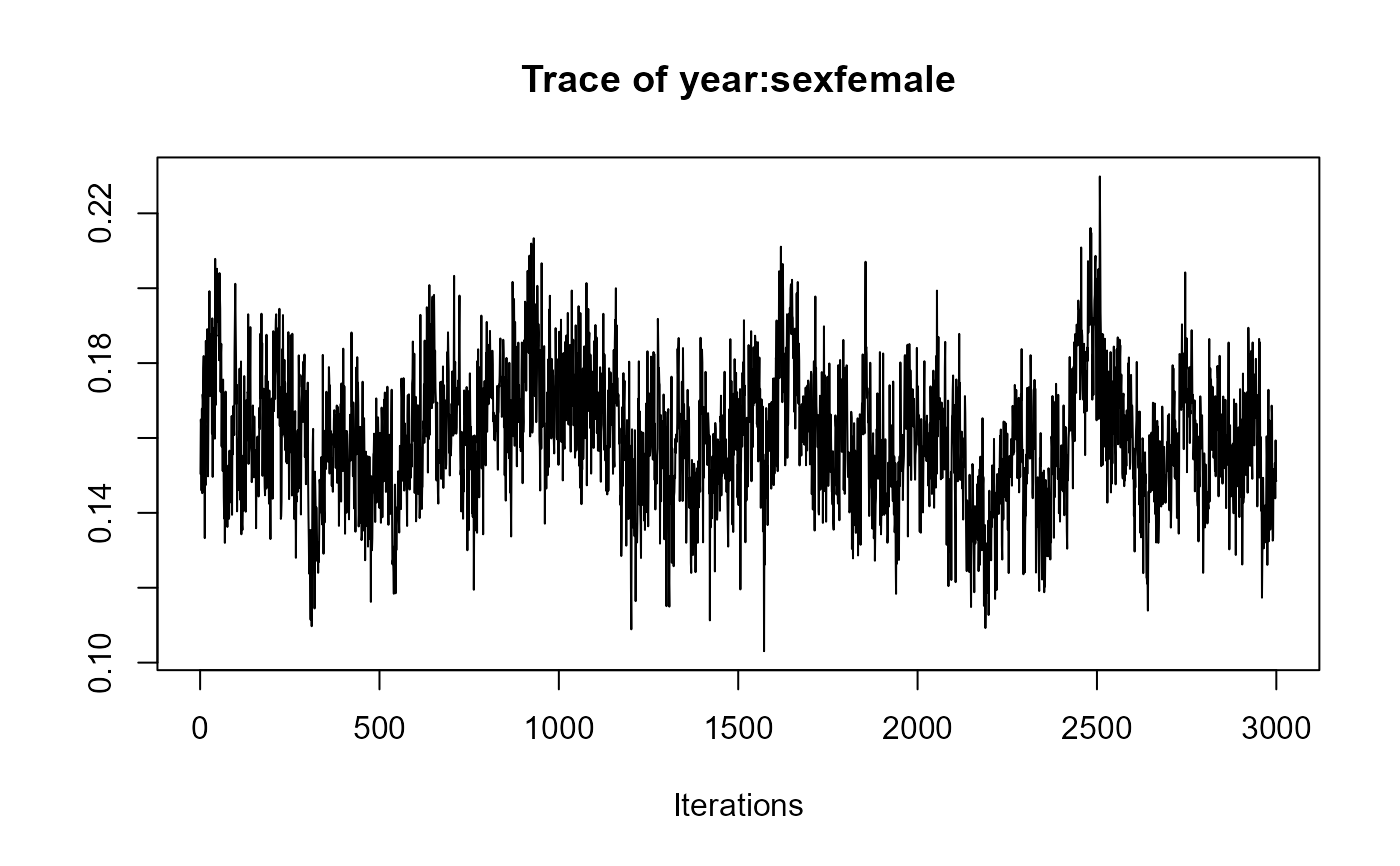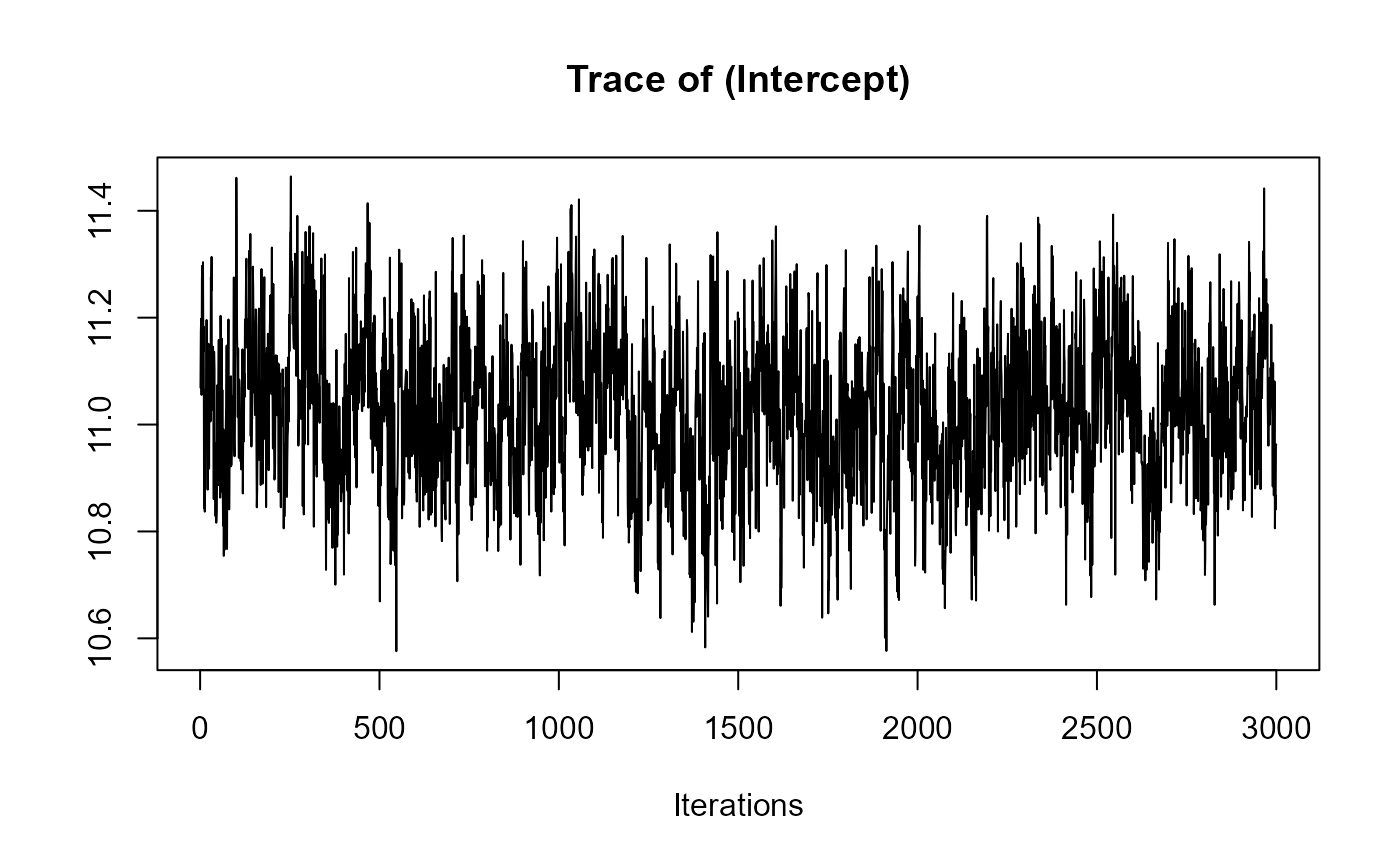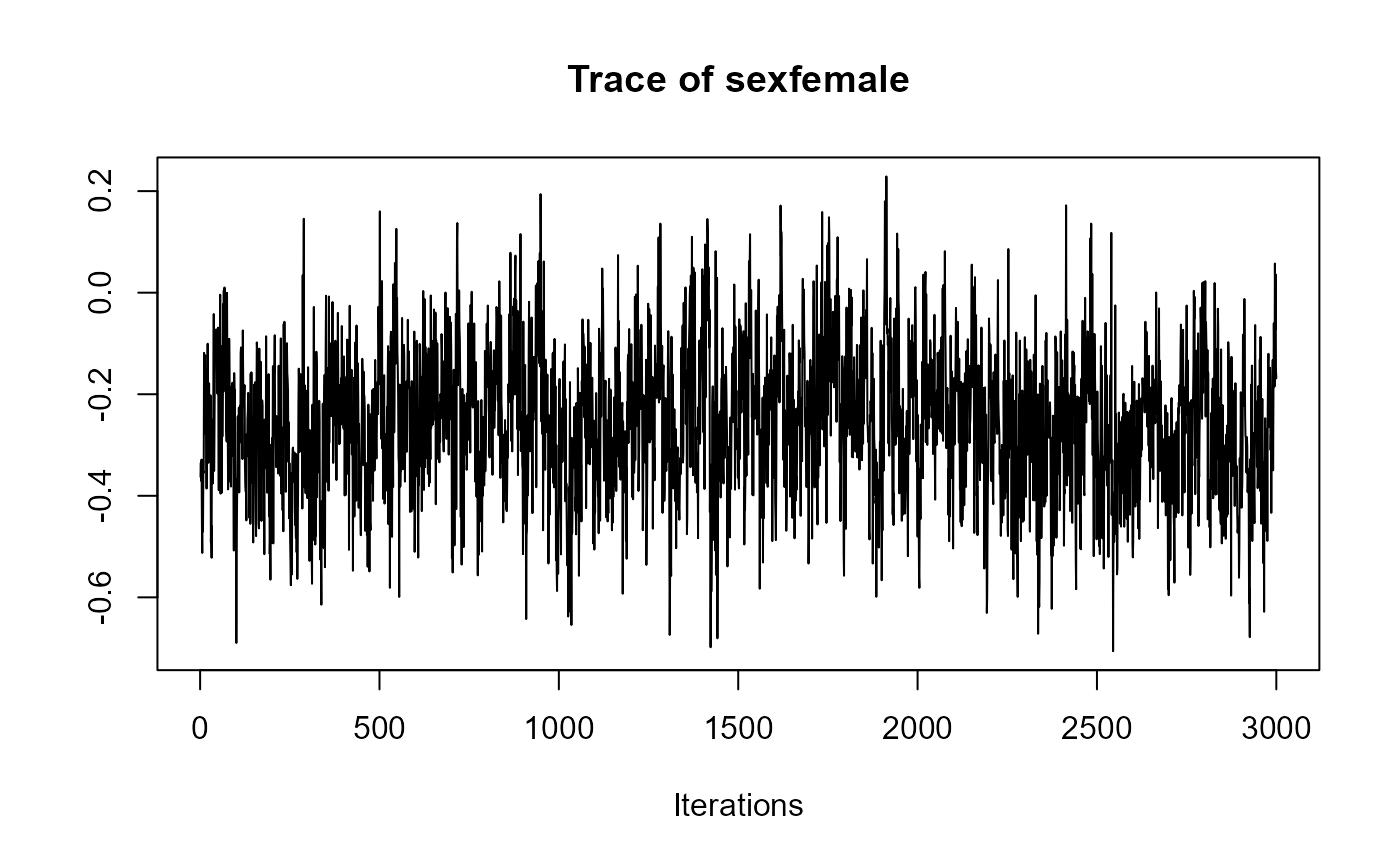# density plot for the fixed effects in the linear mixed submodels
densplot(fit_jm, parm = "betas")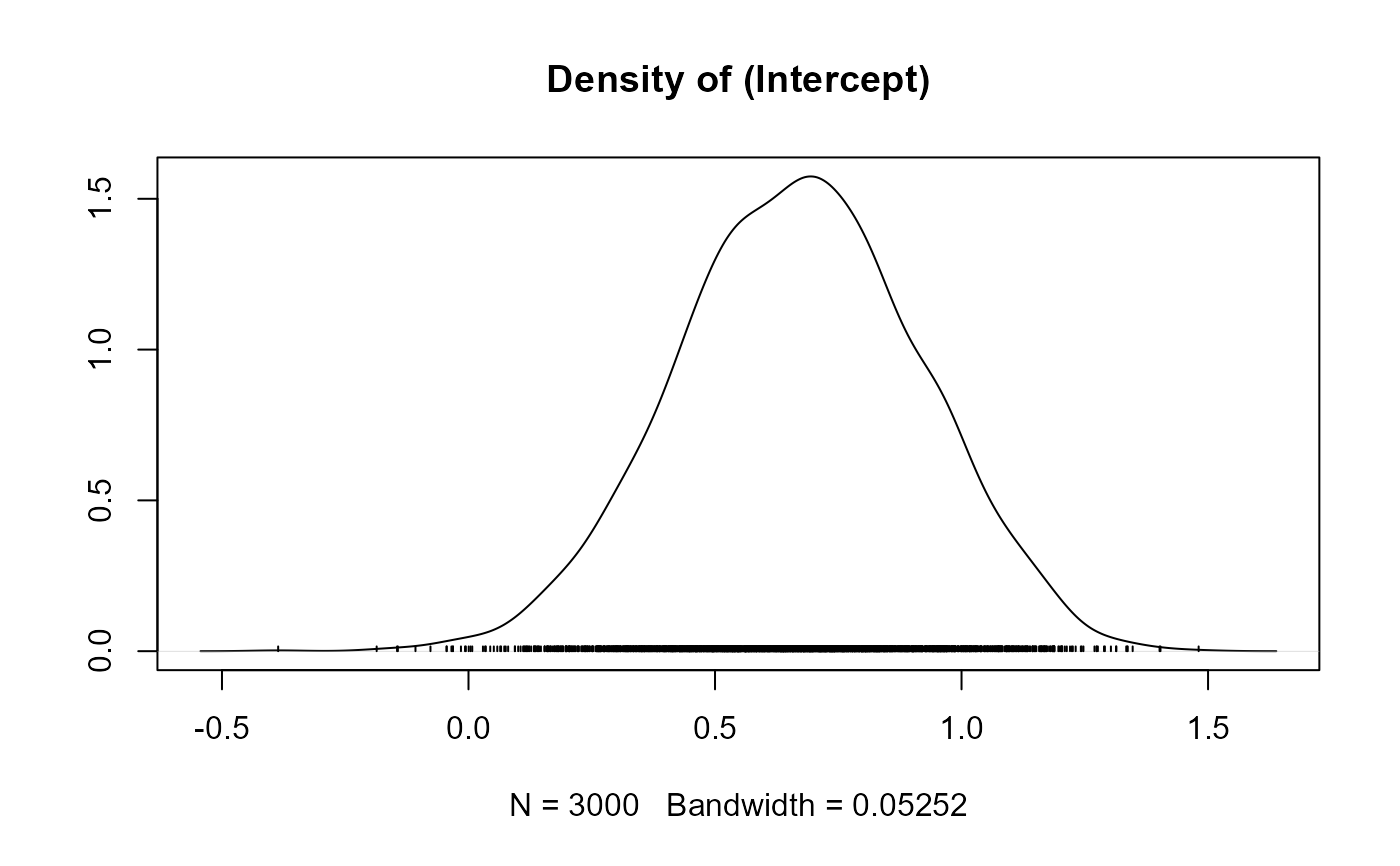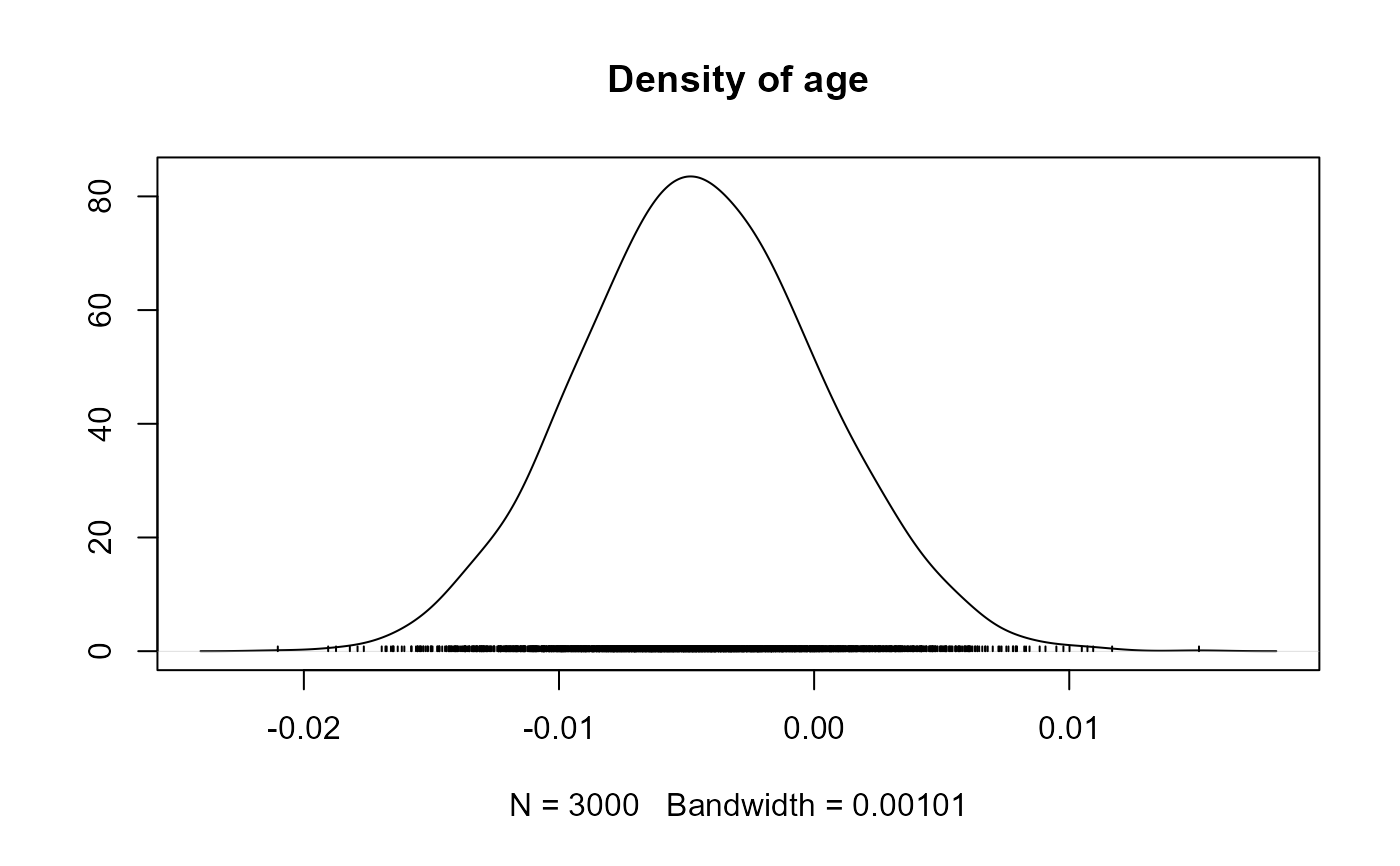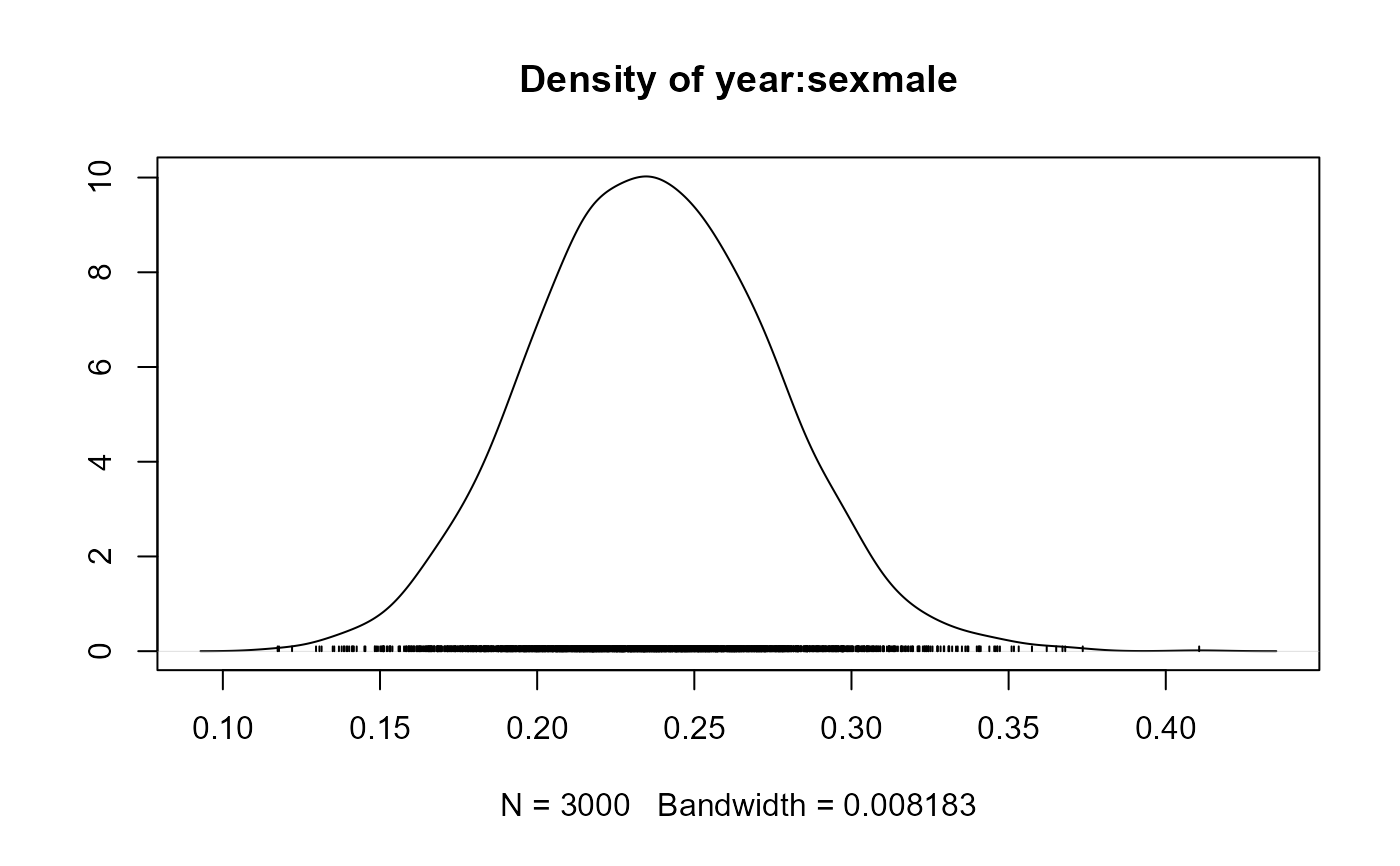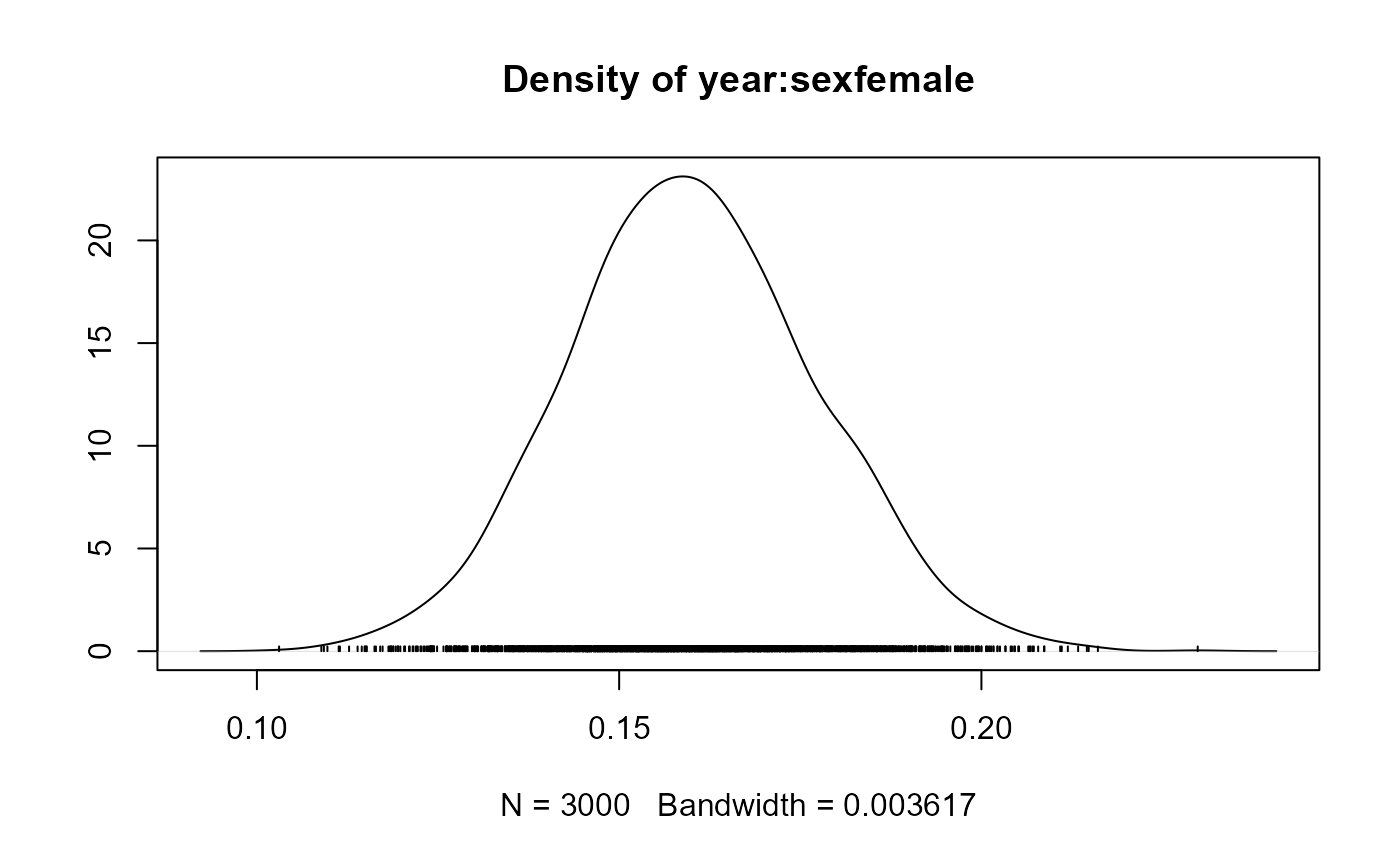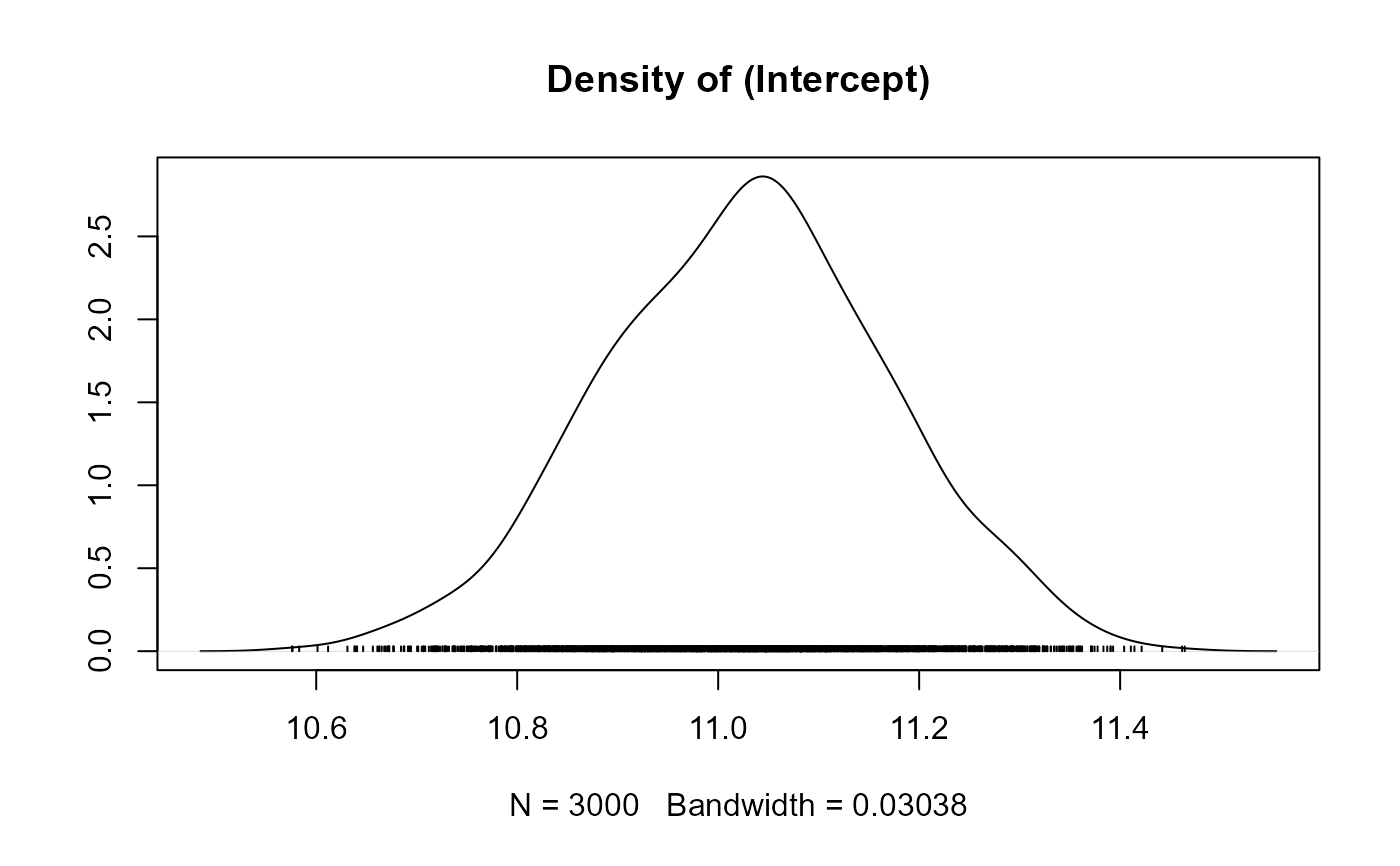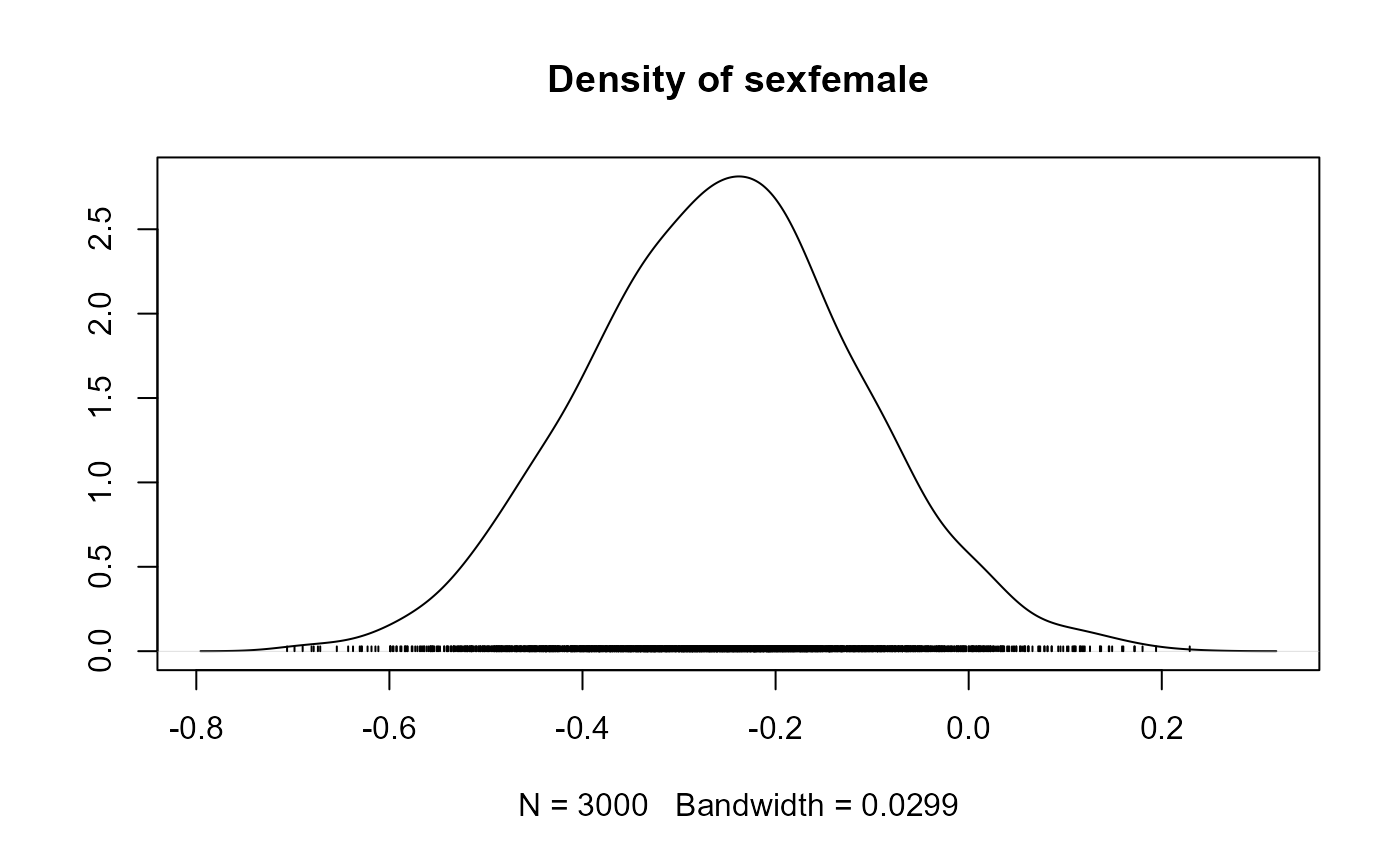# cumulative quantile plot for the fixed effects in the linear mixed submodels
cumuplot(fit_jm, parm = "betas")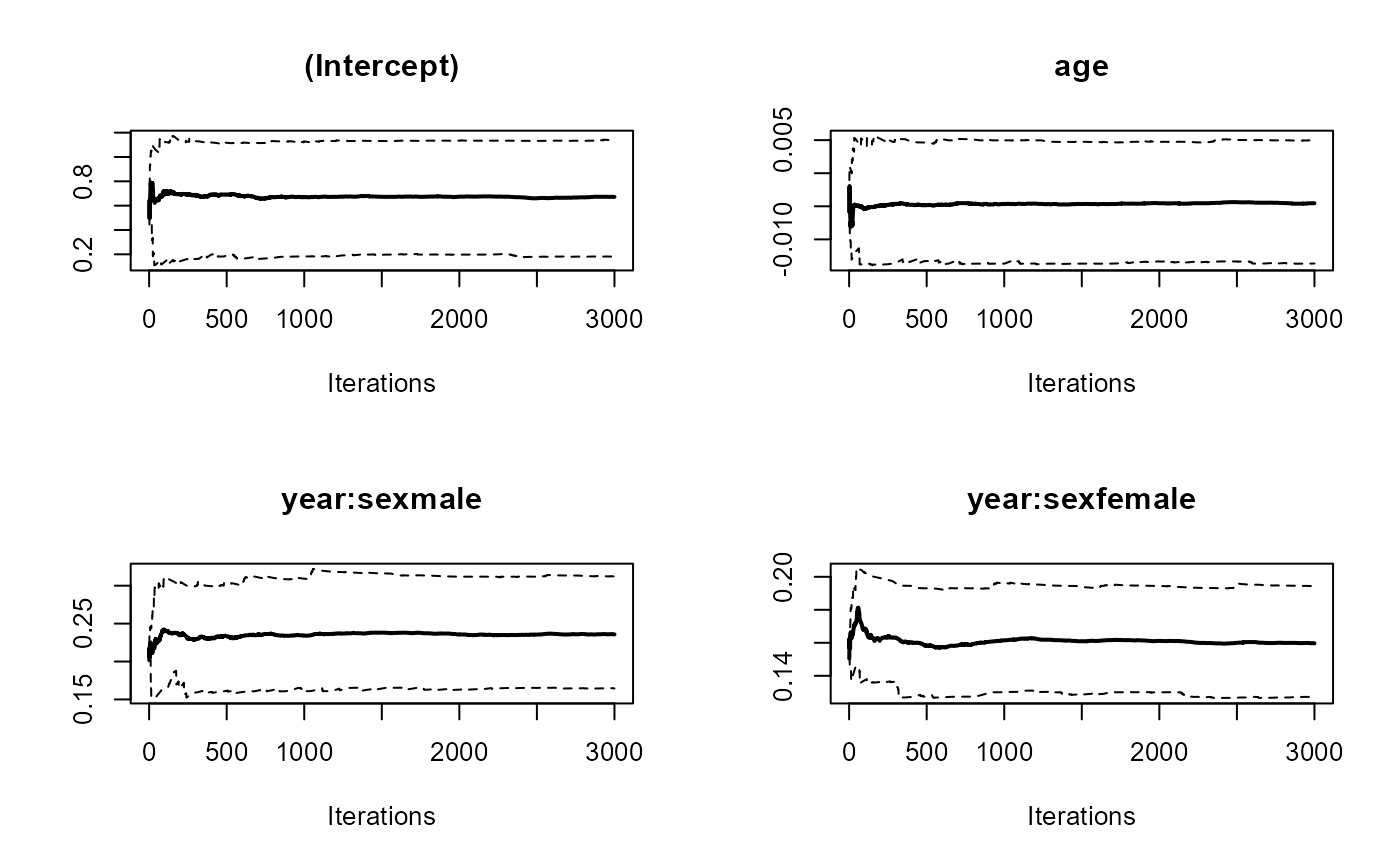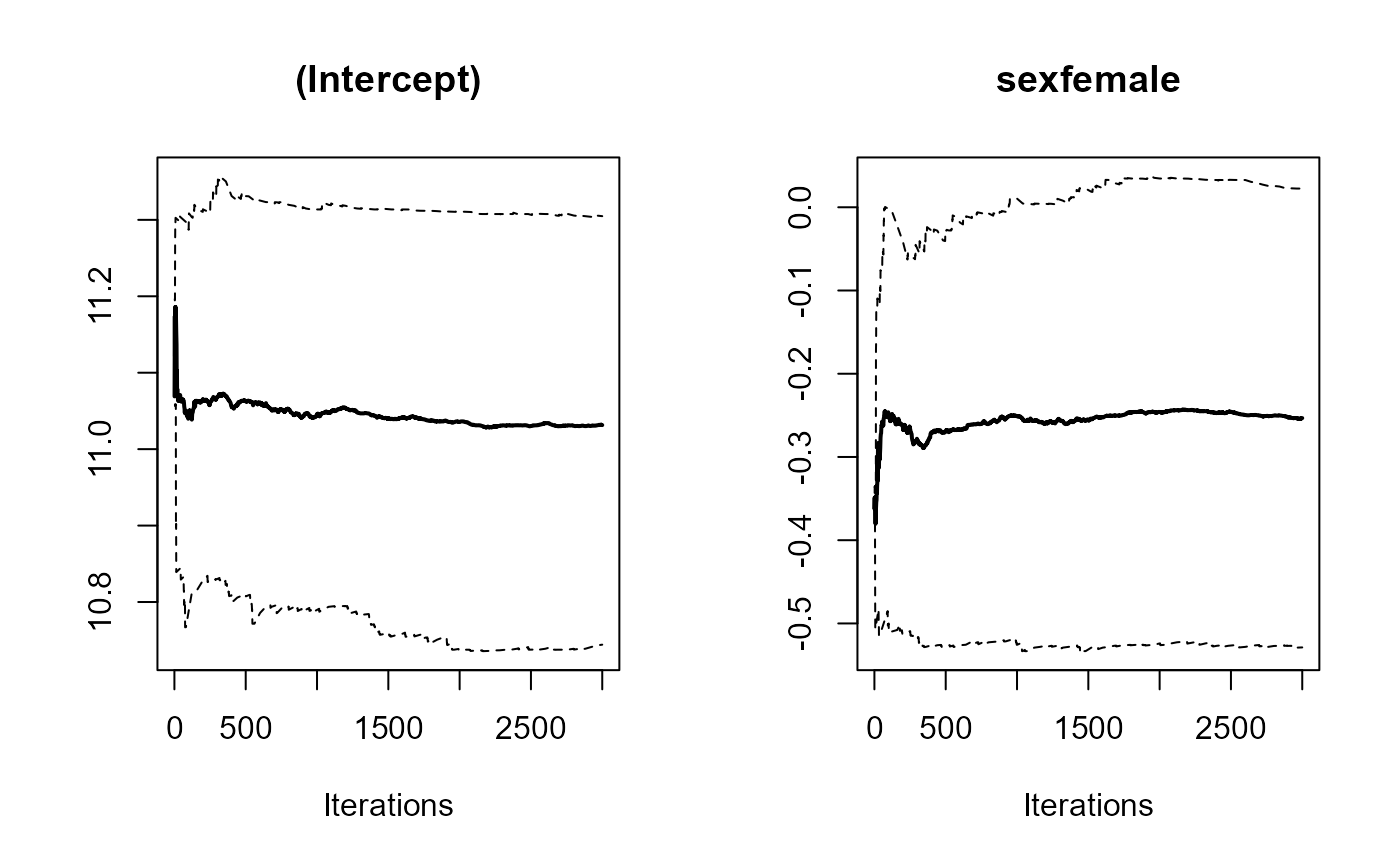# trace plot for the fixed effects in the linear mixed submodels
ggtraceplot(fit_jm, parm = "betas")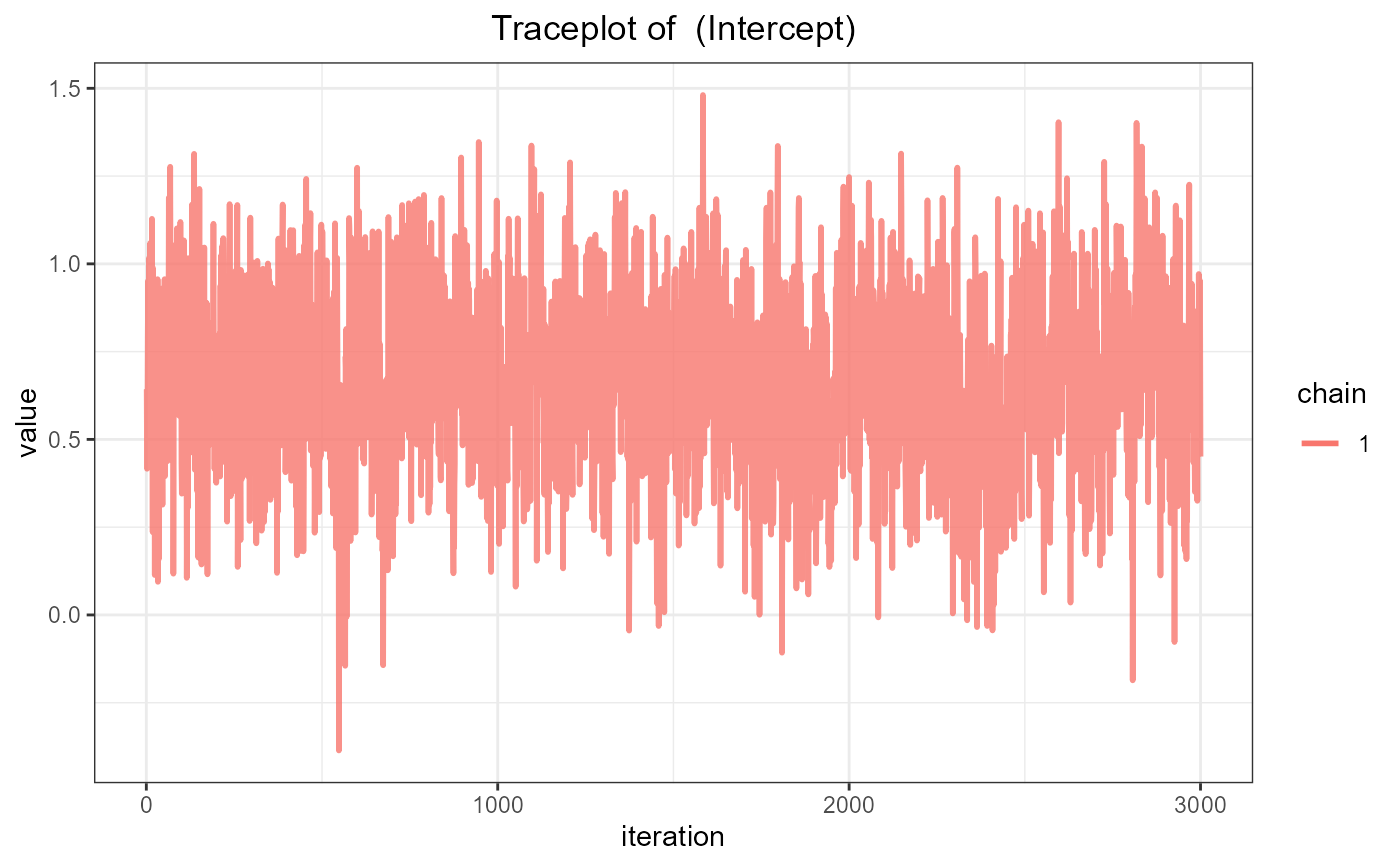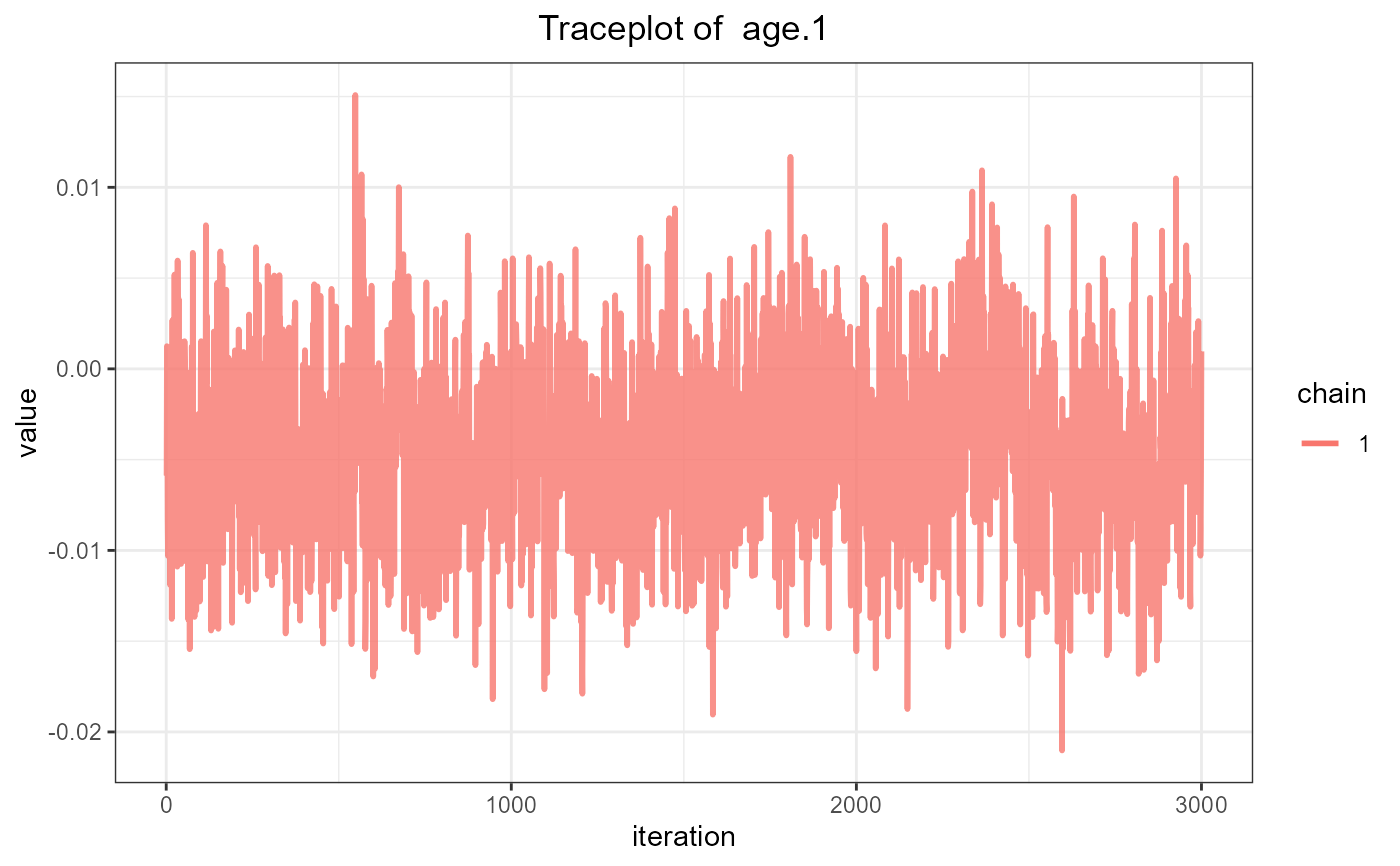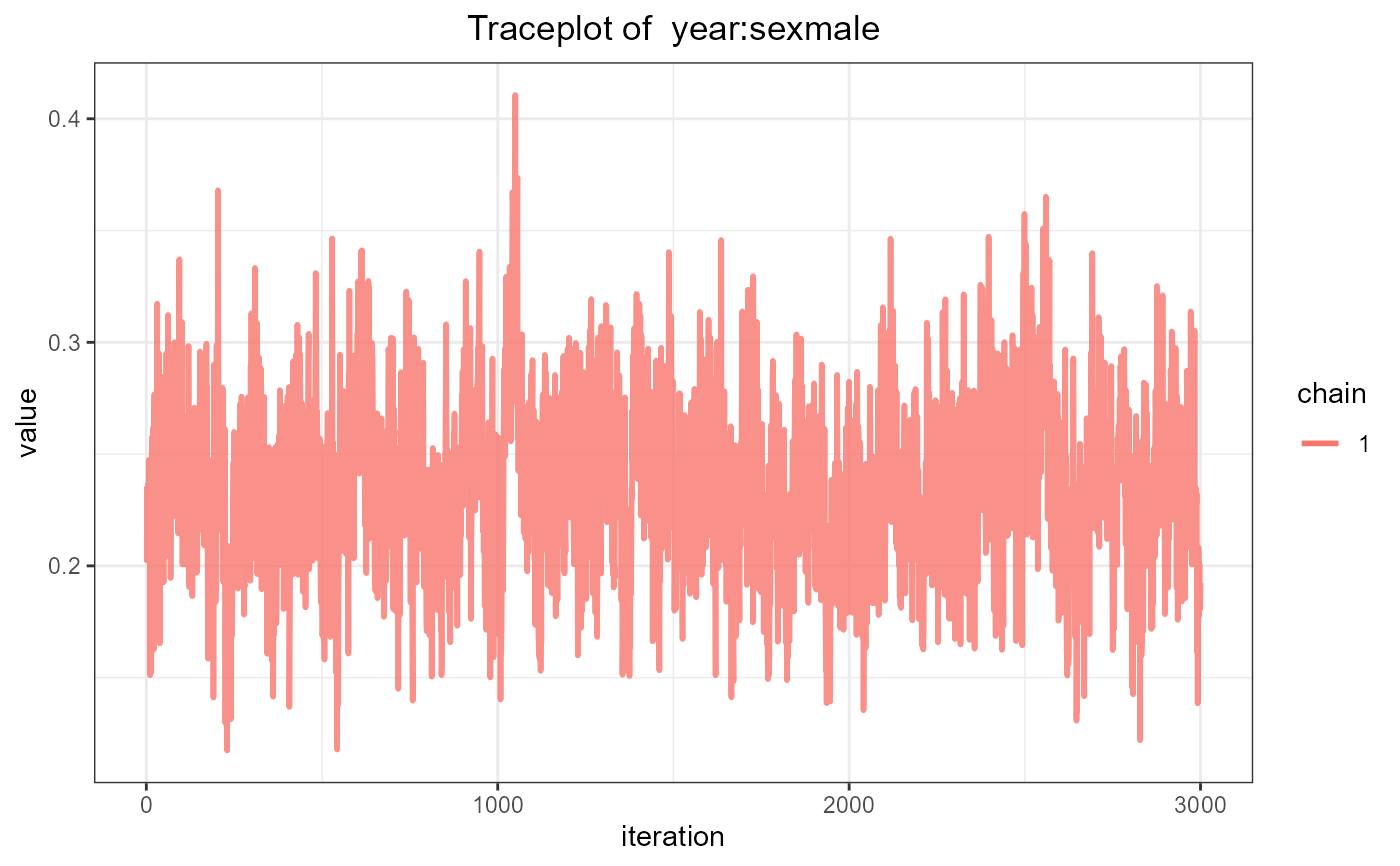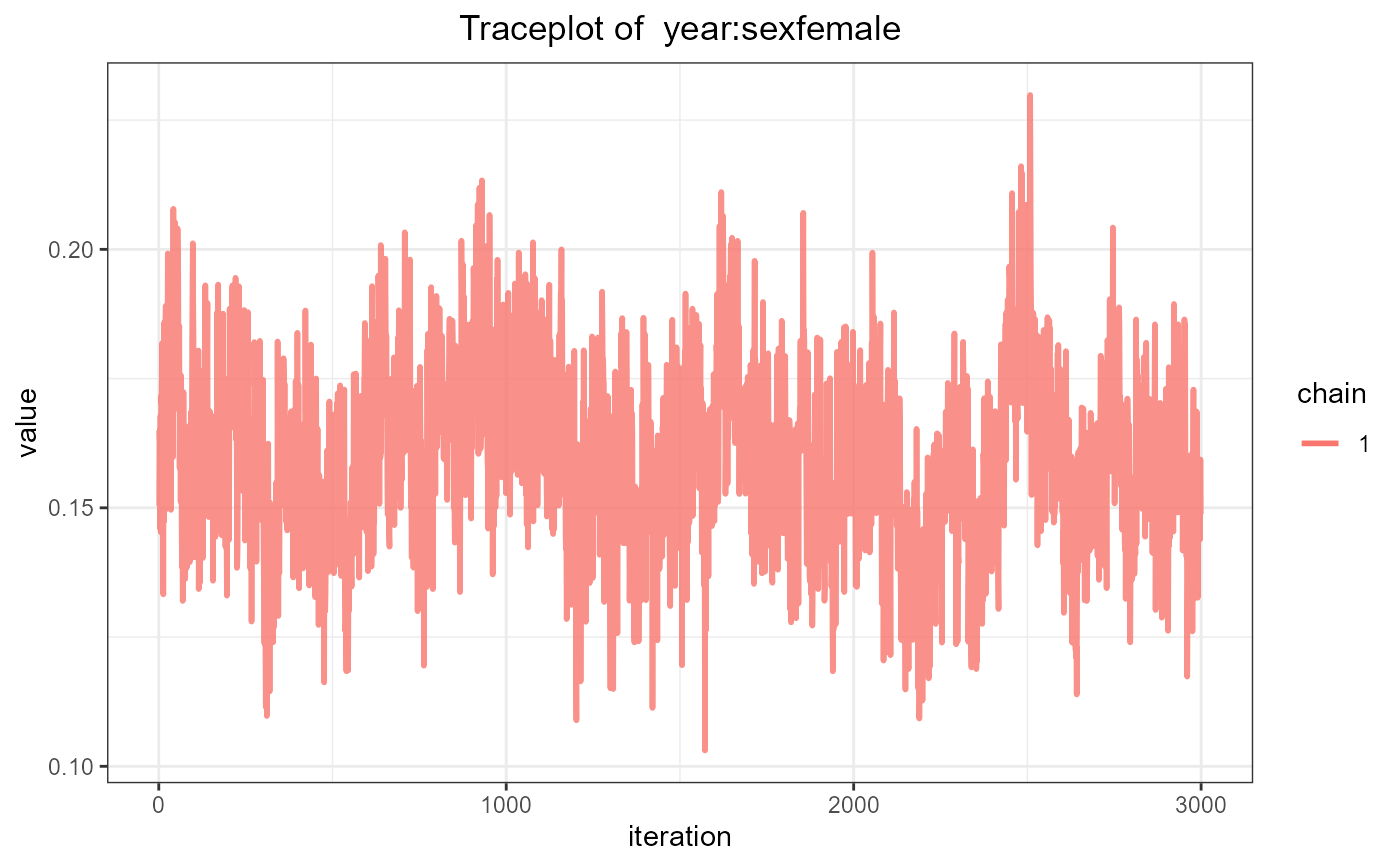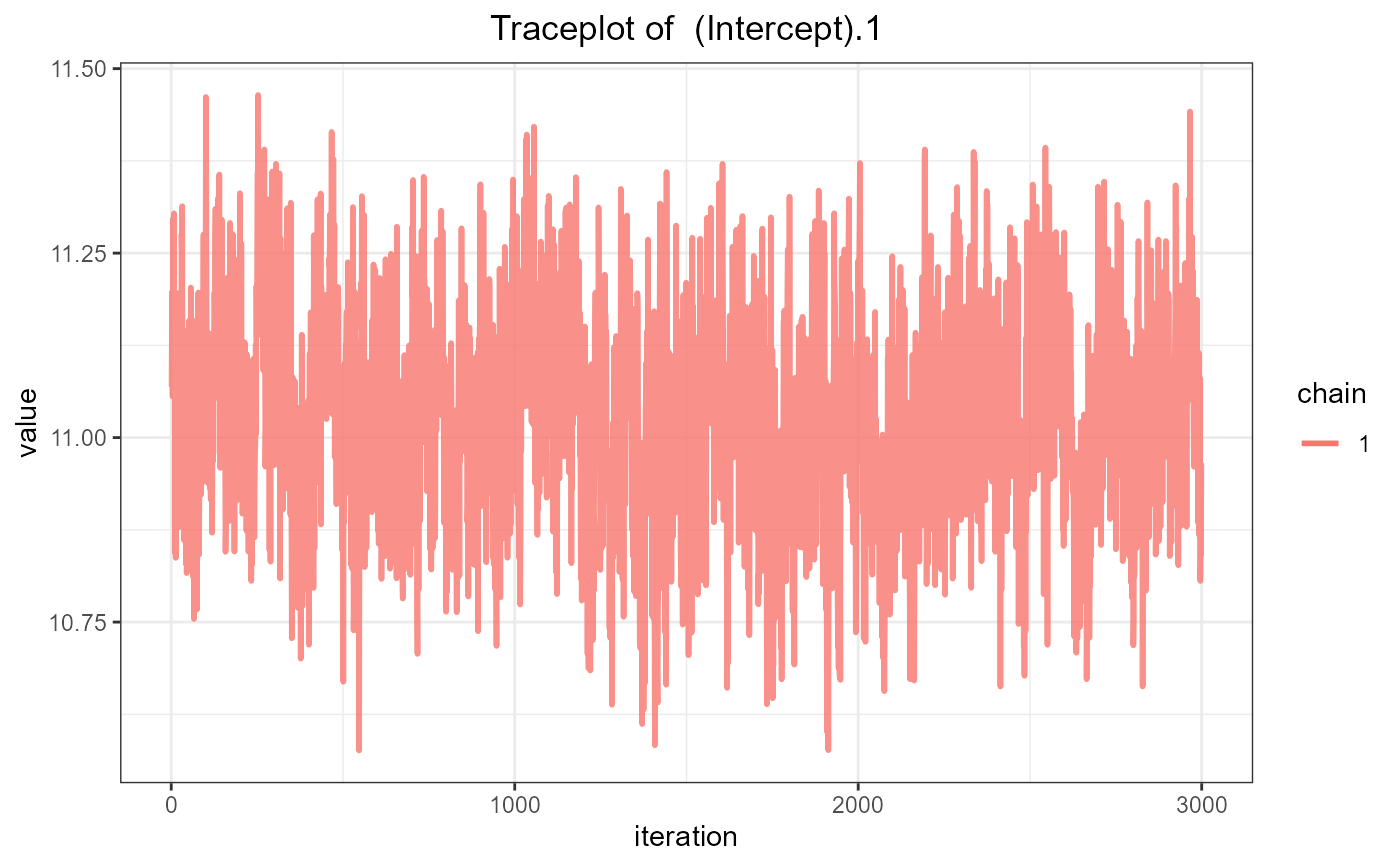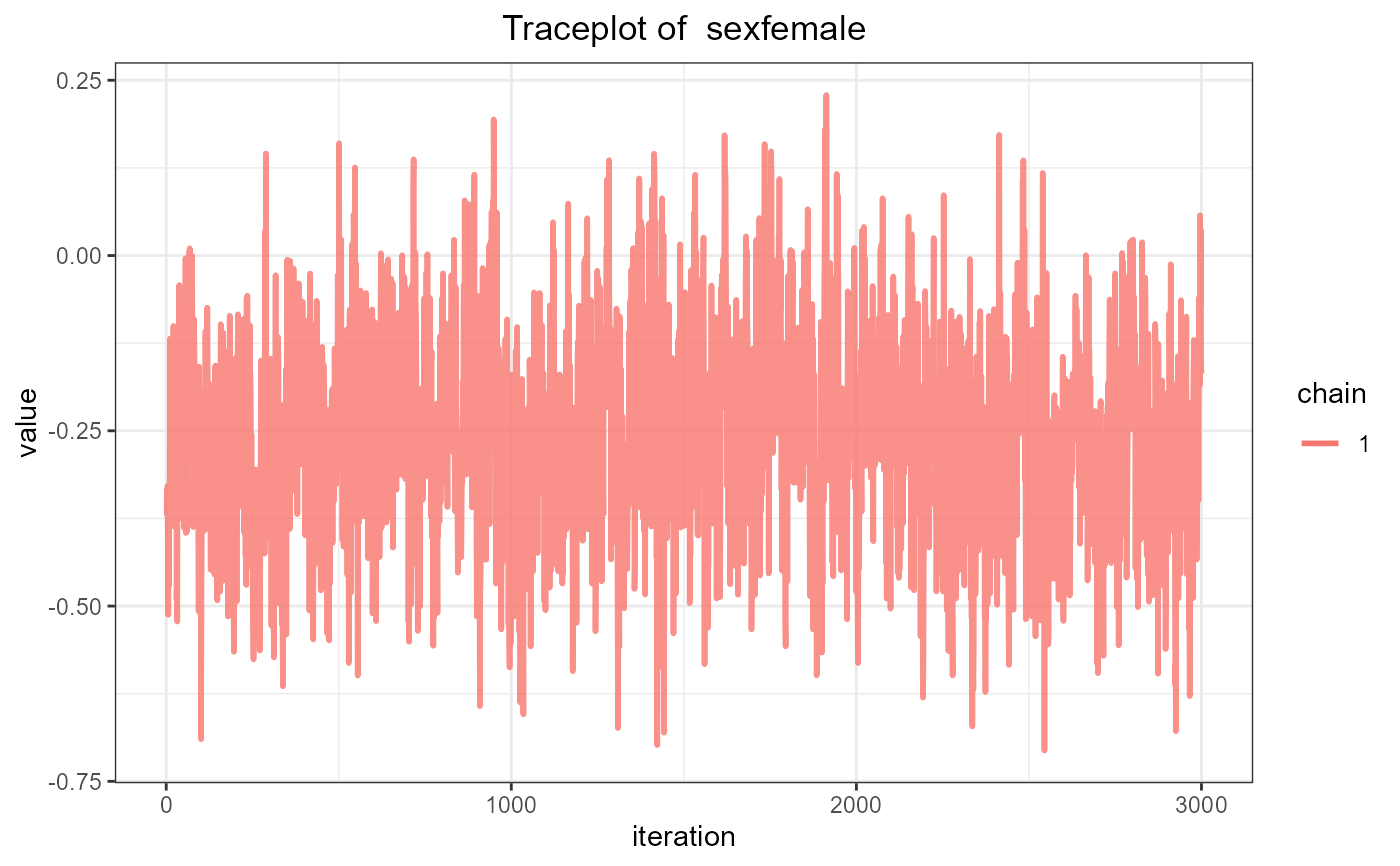ggtraceplot(fit_jm, parm = "betas", grid = TRUE)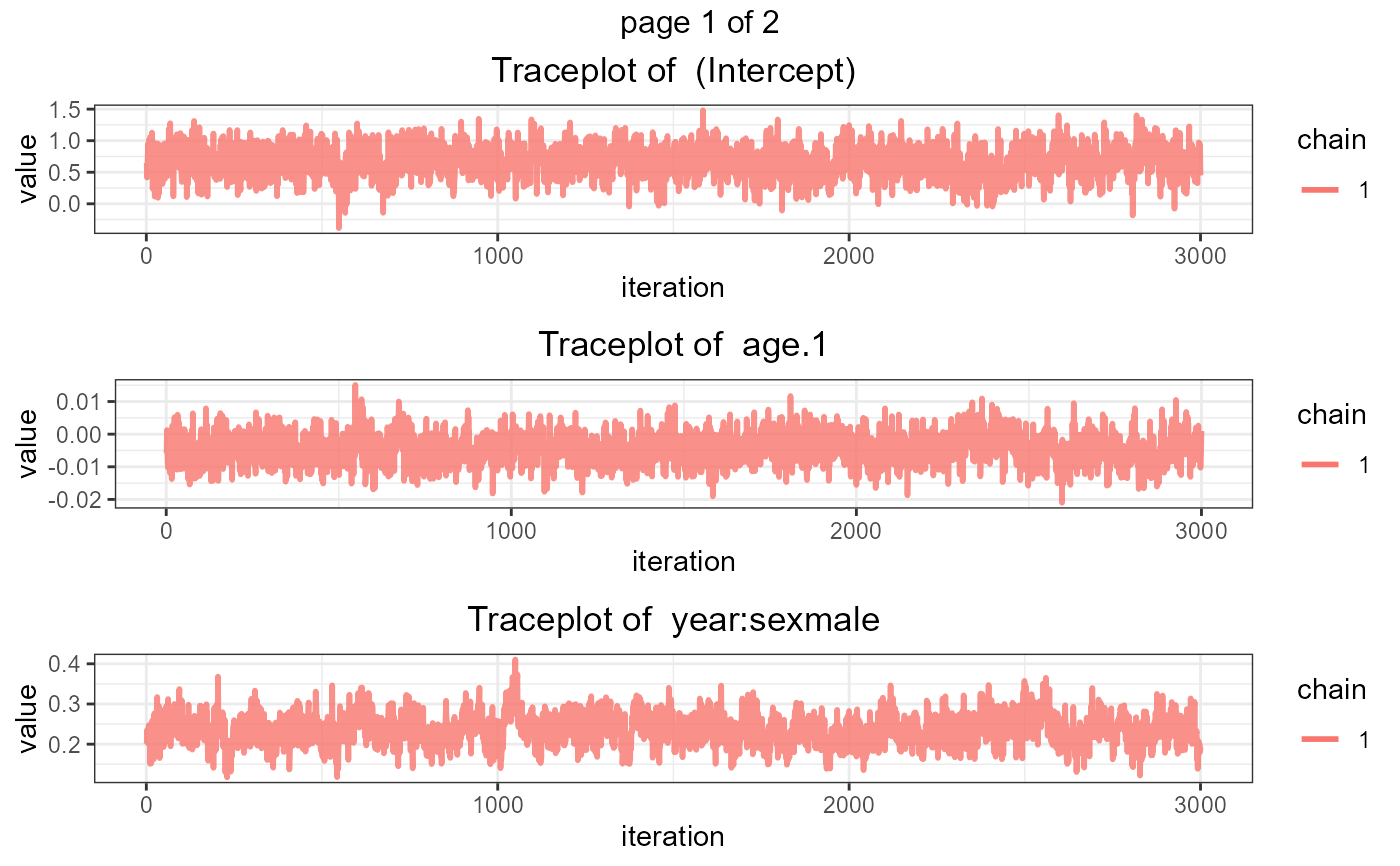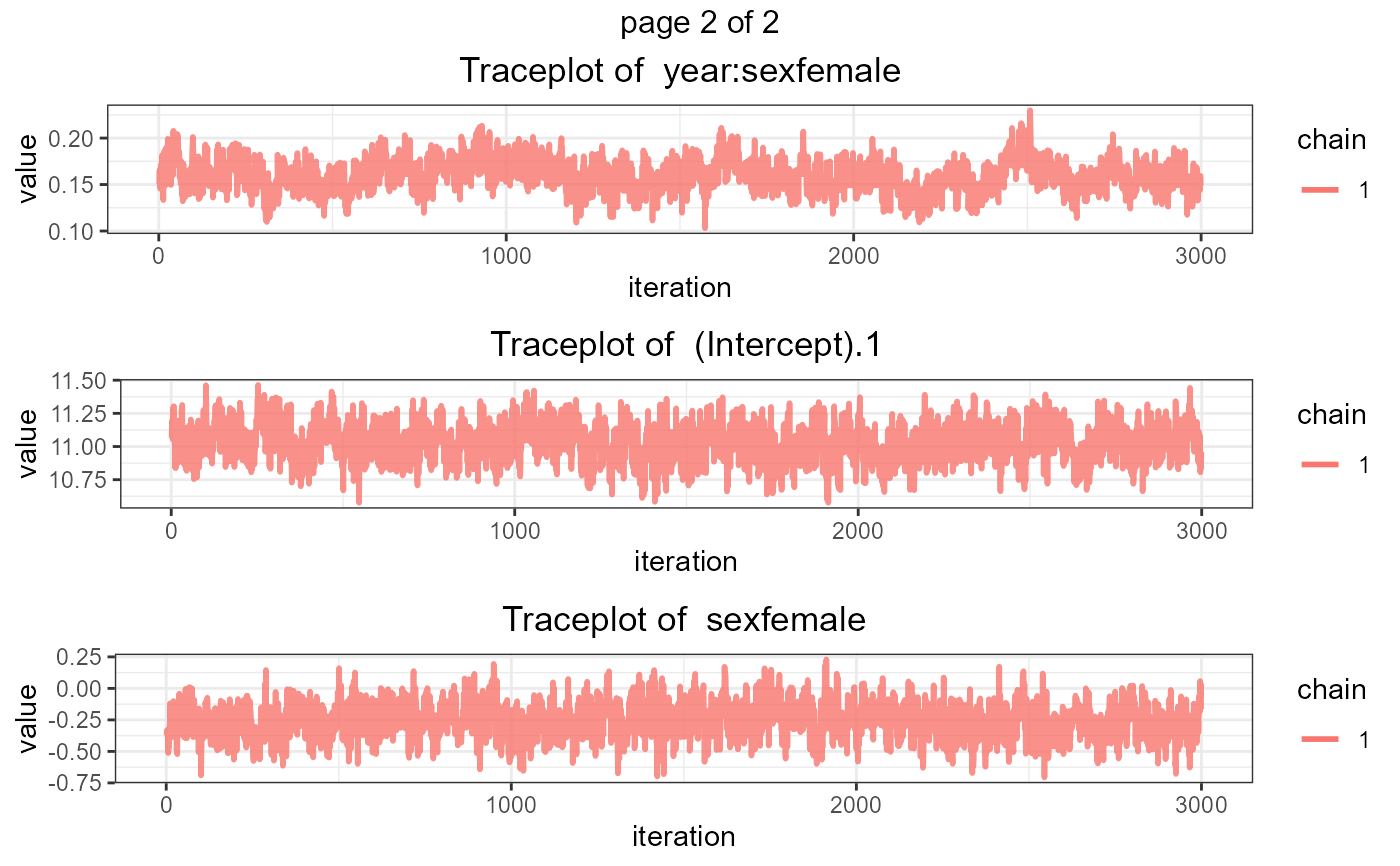ggtraceplot(fit_jm, parm = "betas", custom_theme = c('1' = 'black'))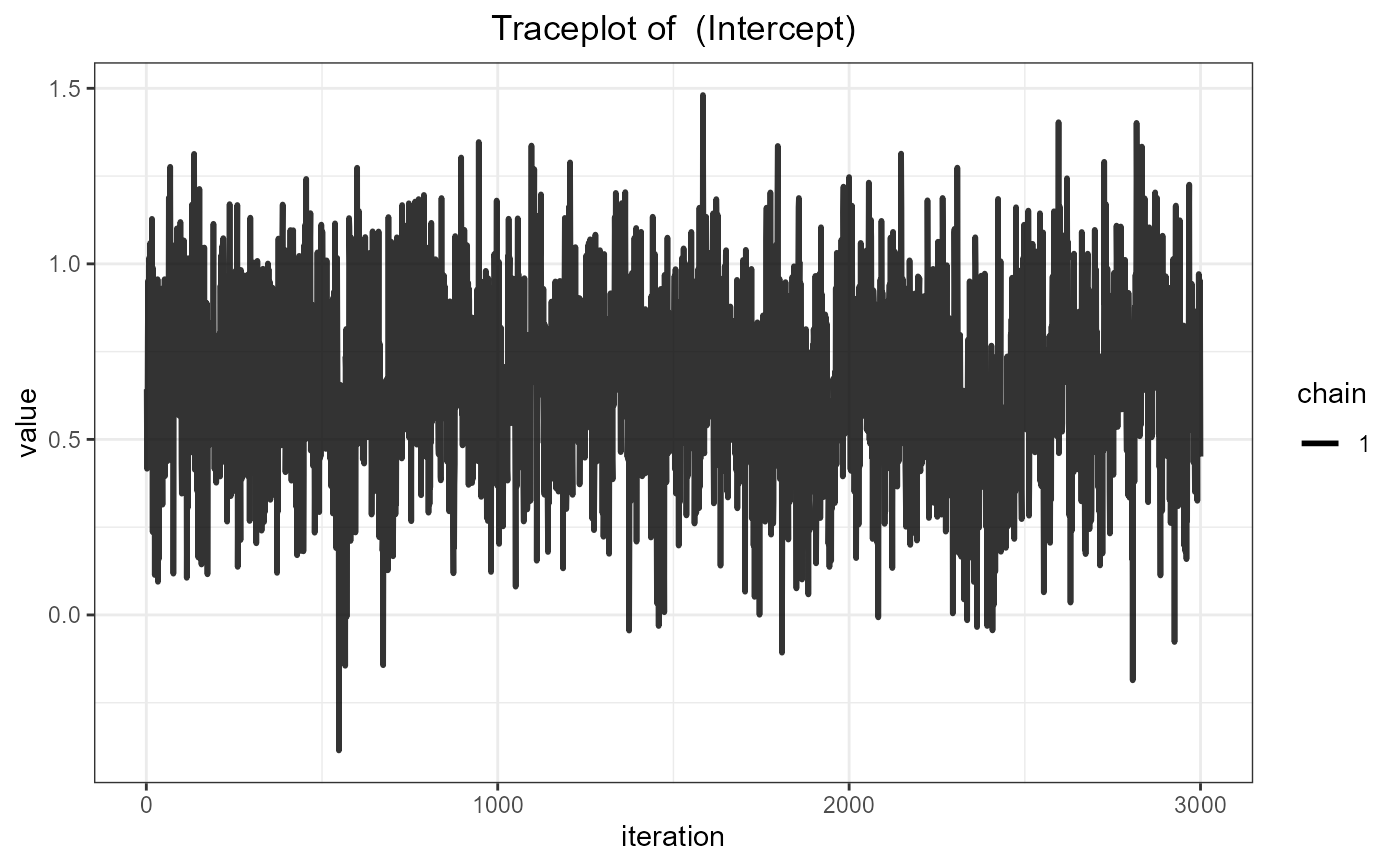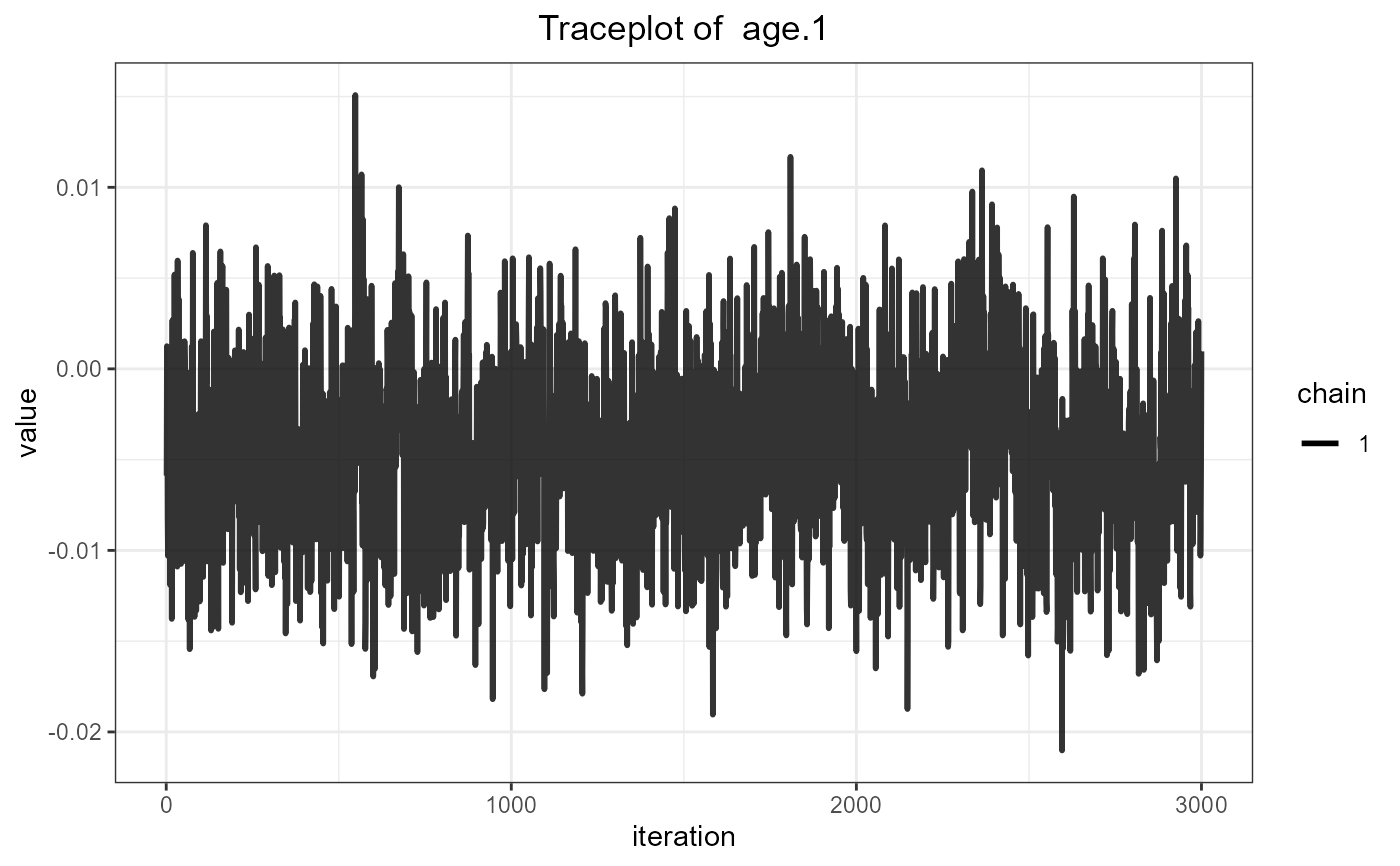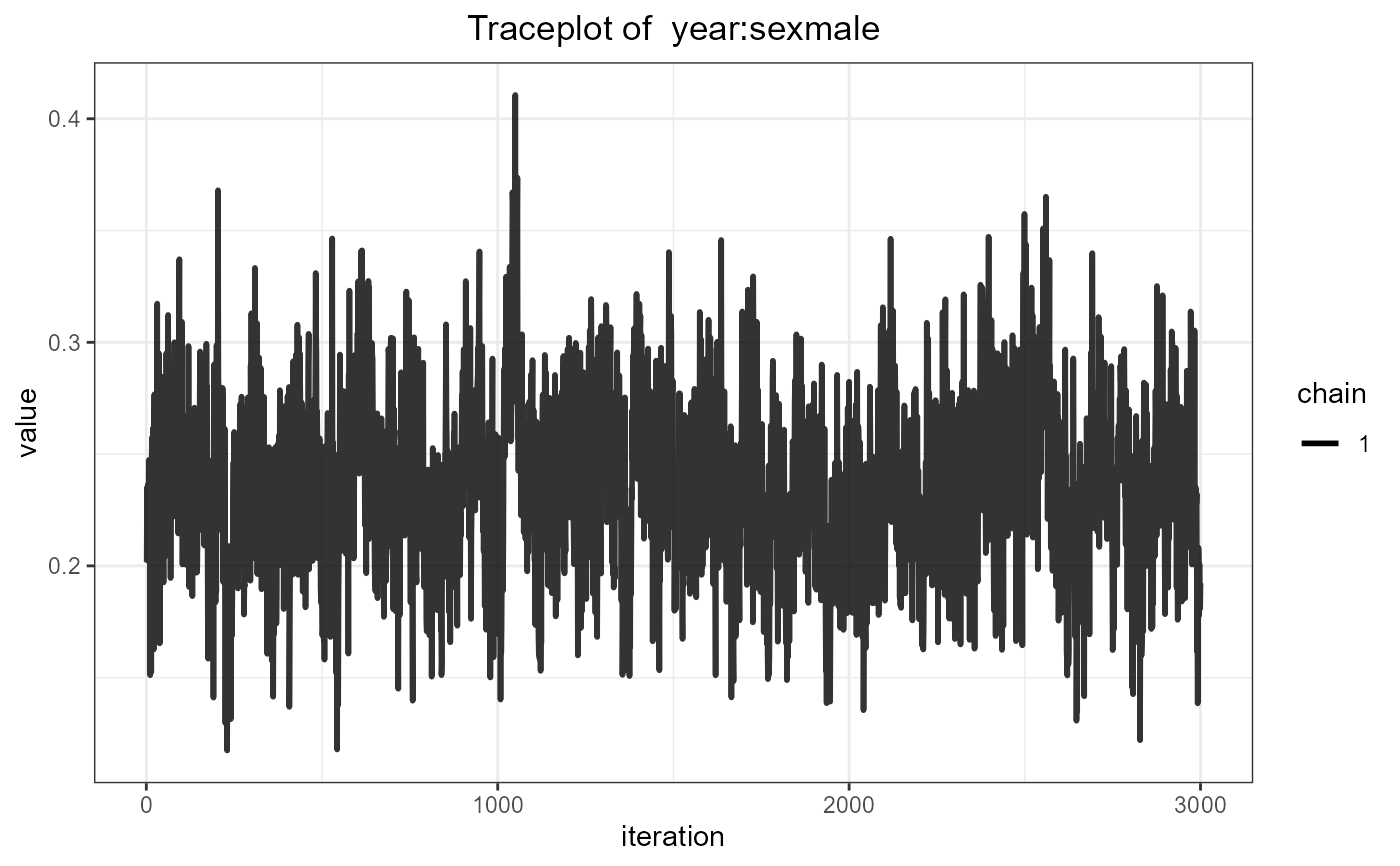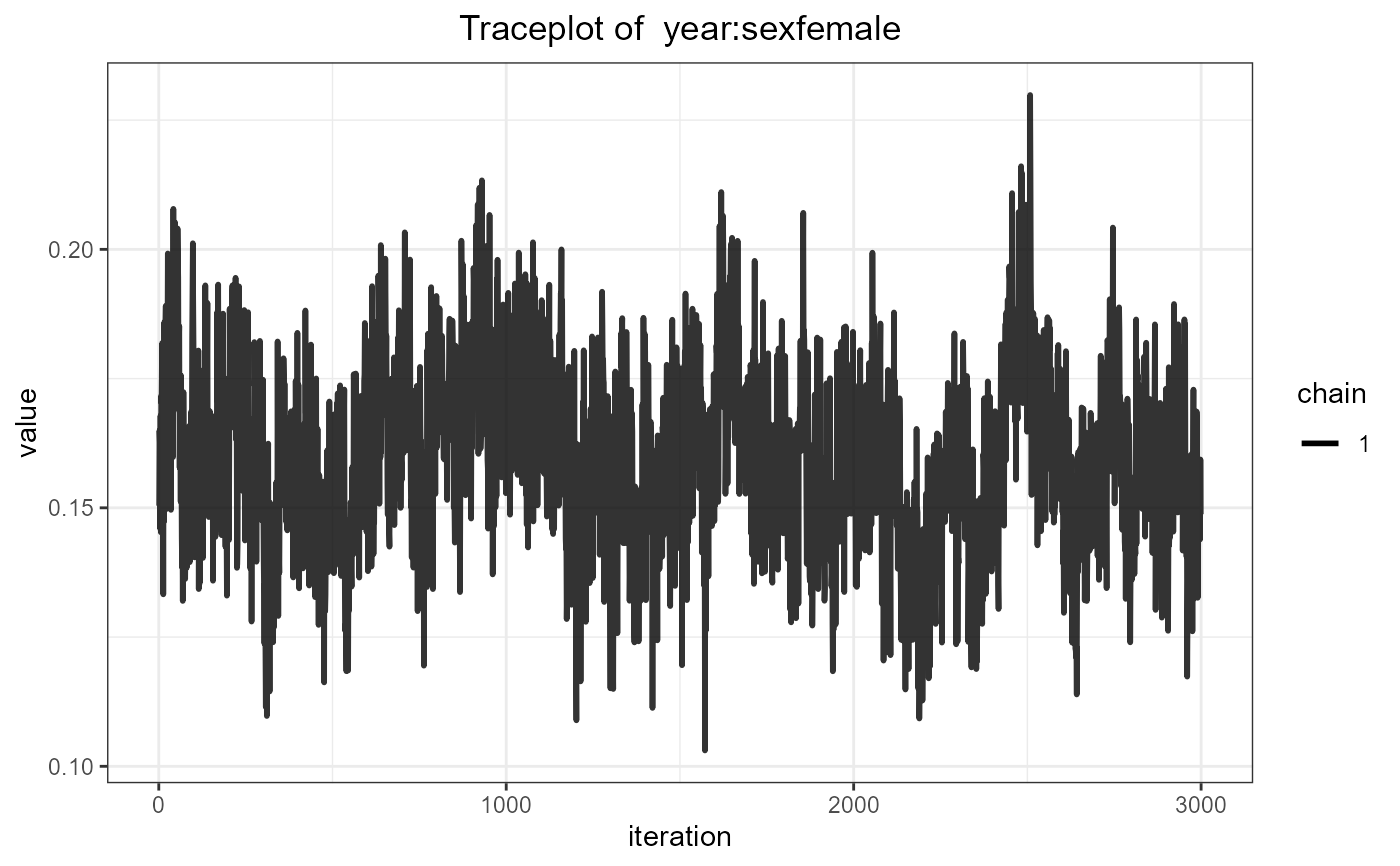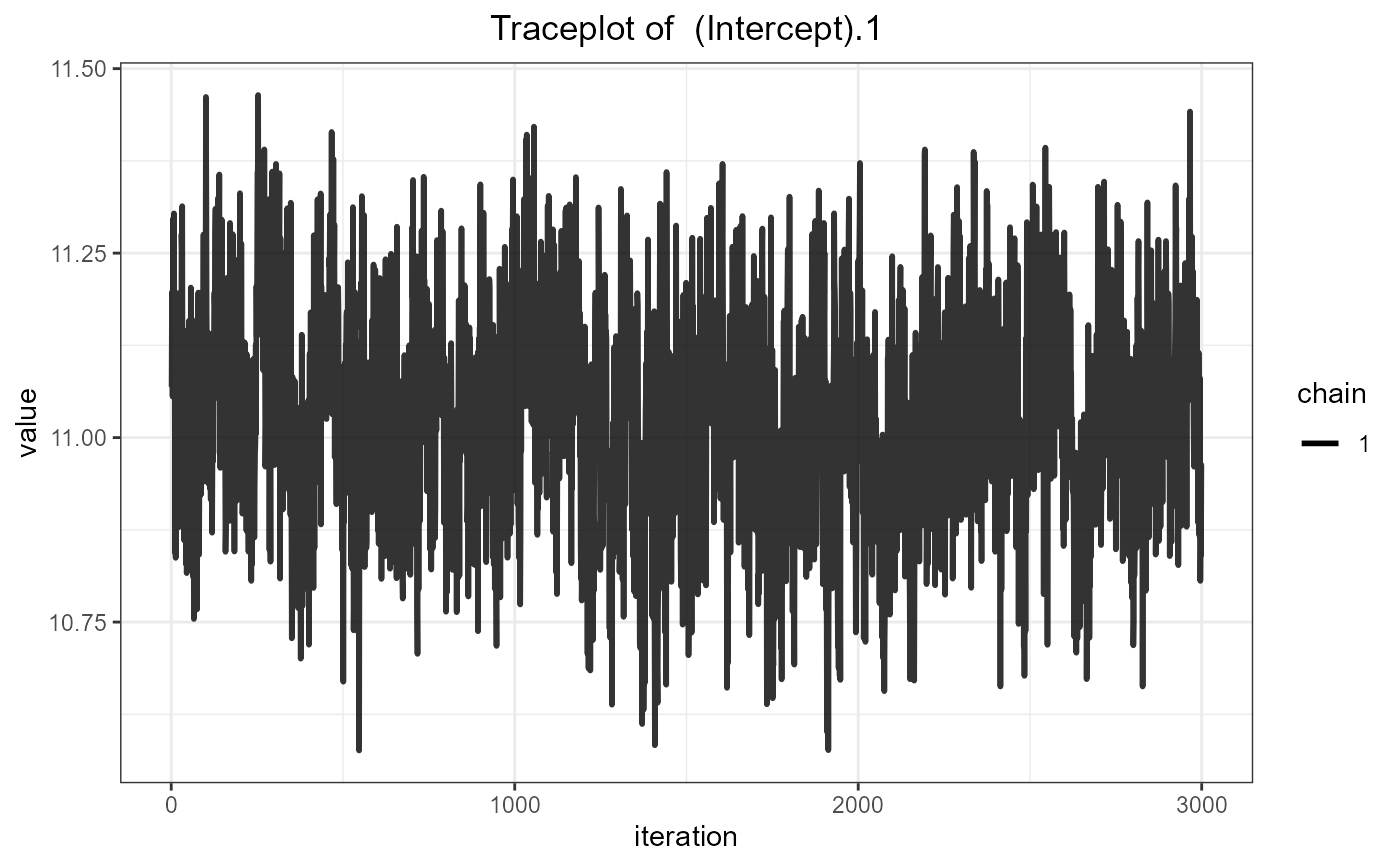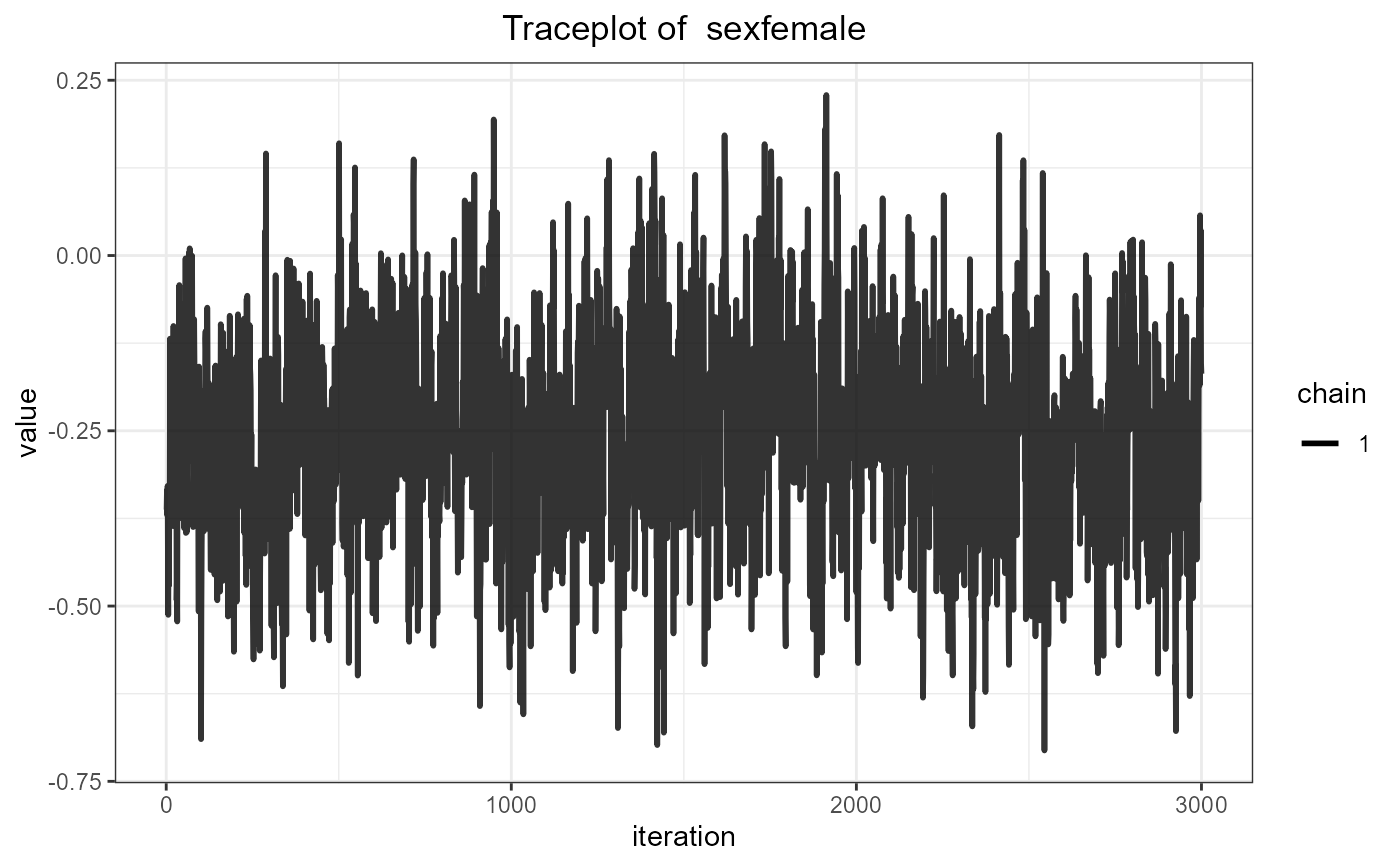# trace plot for the fixed effects in the linear mixed submodels
ggdensityplot(fit_jm, parm = "betas")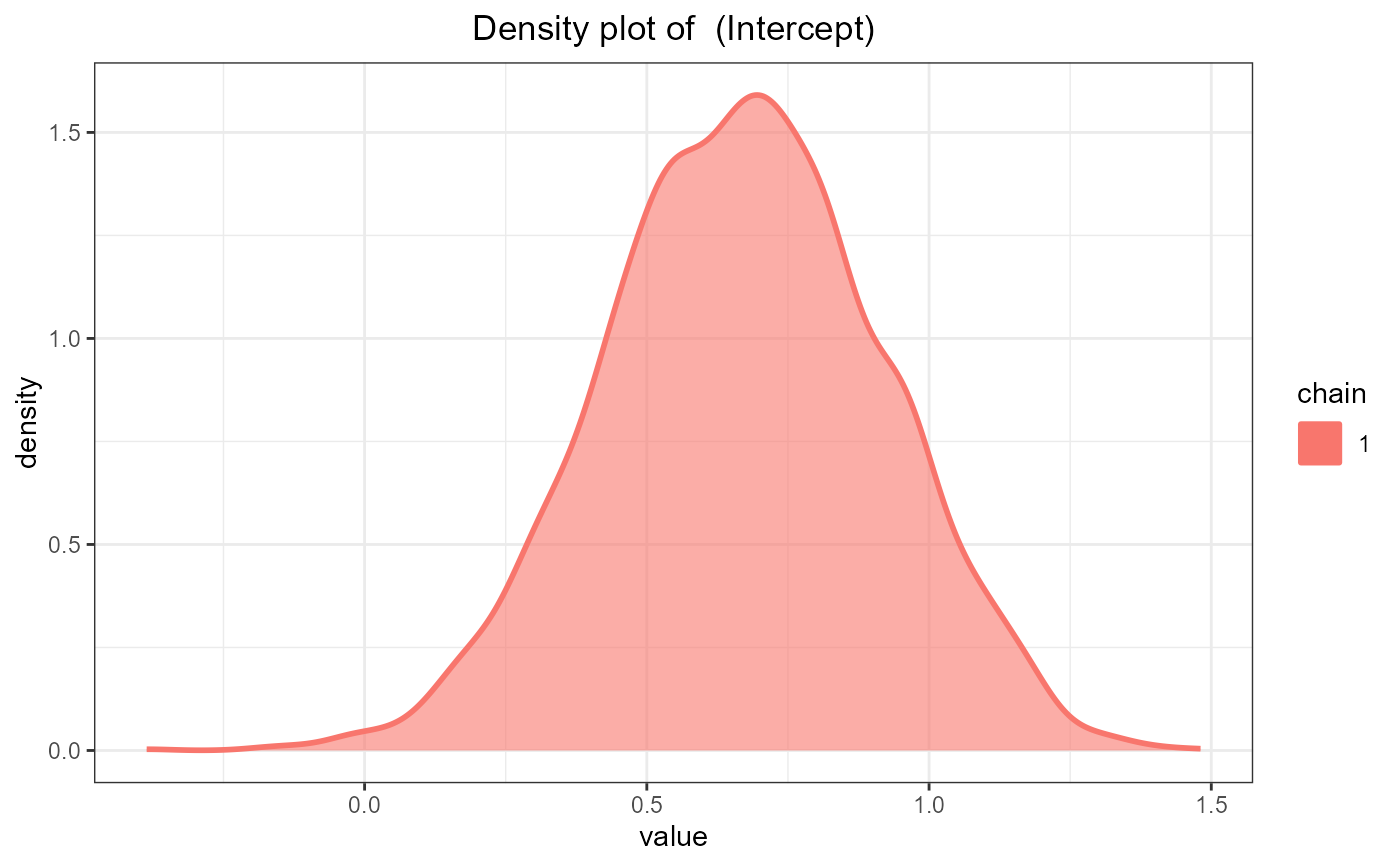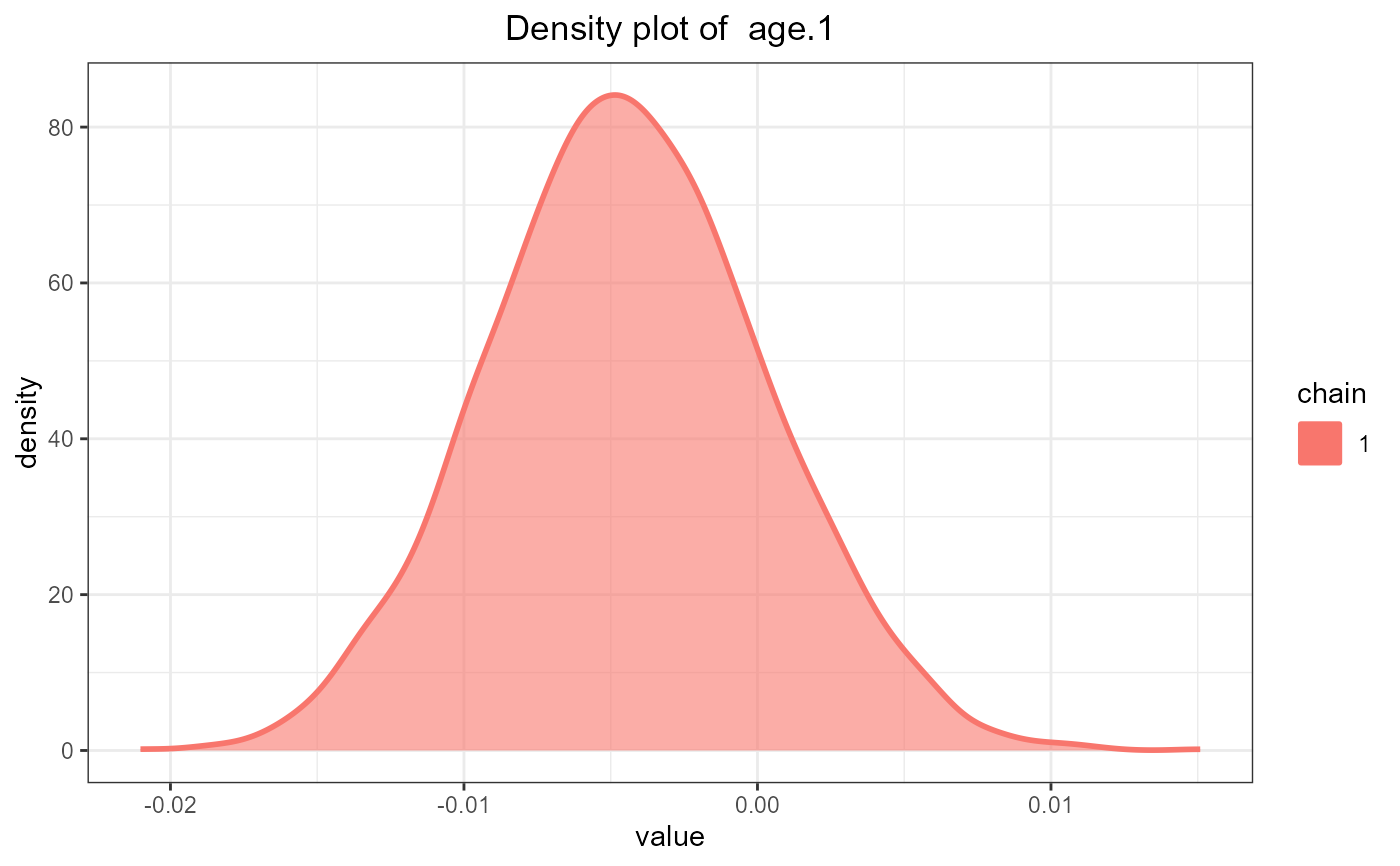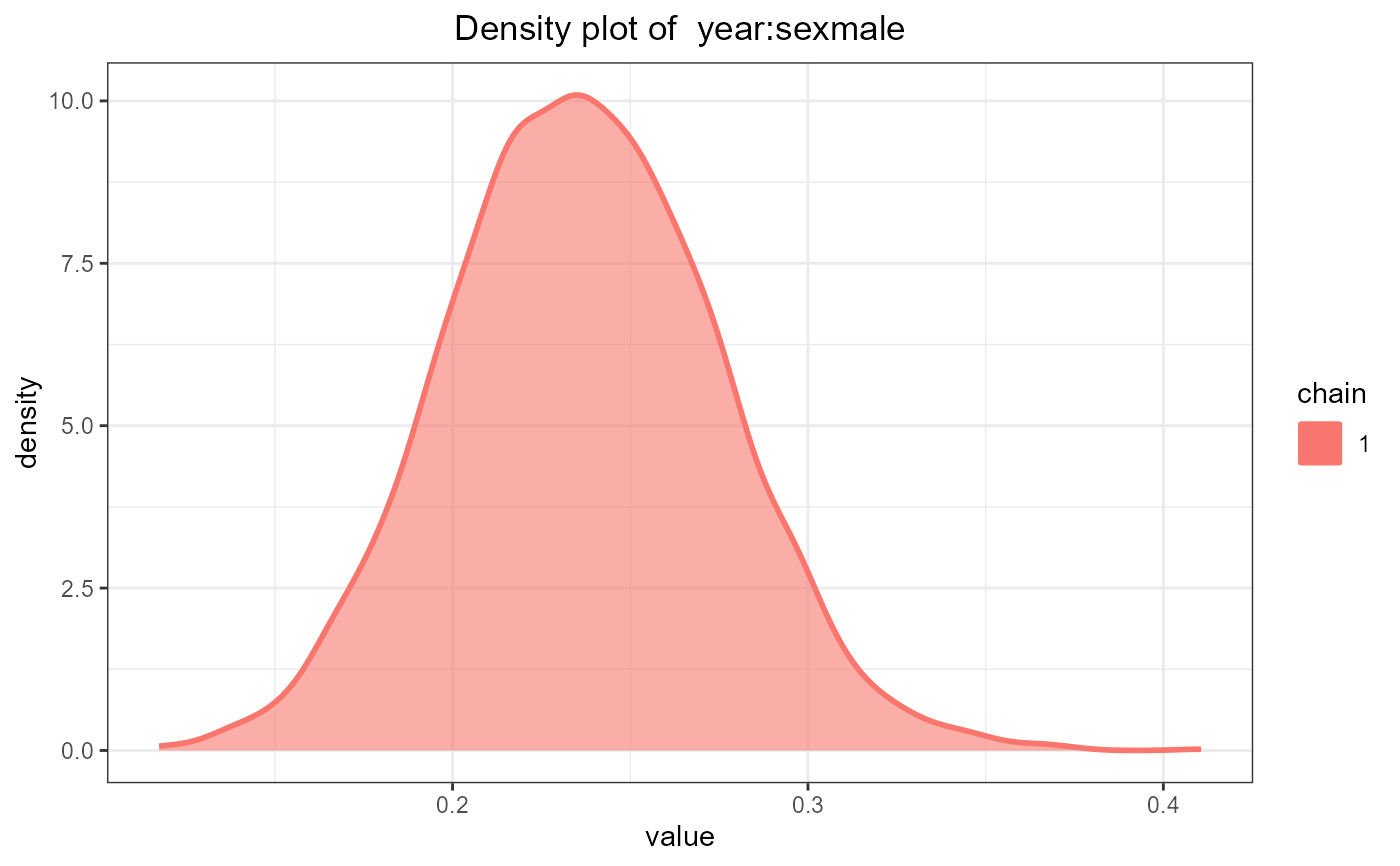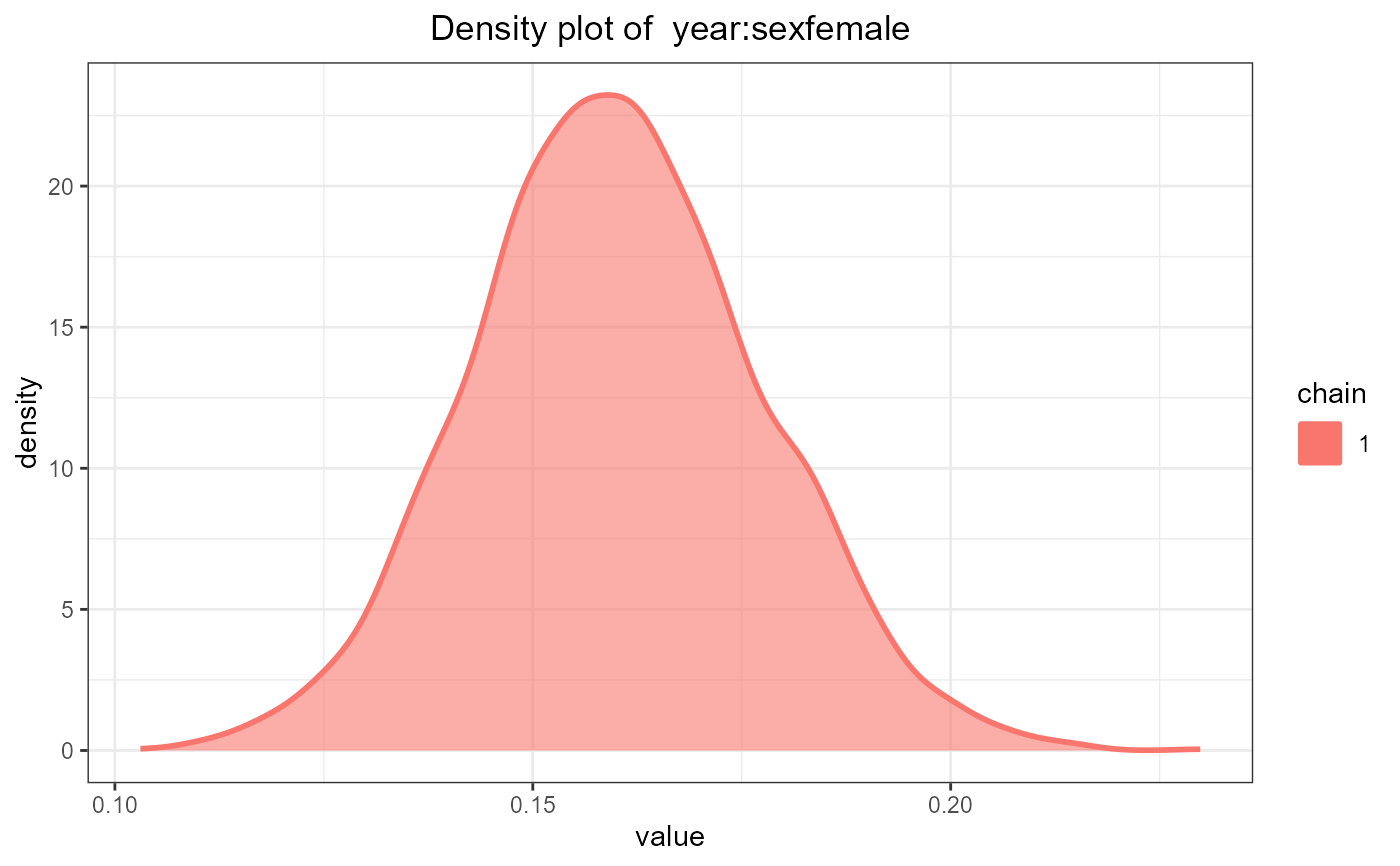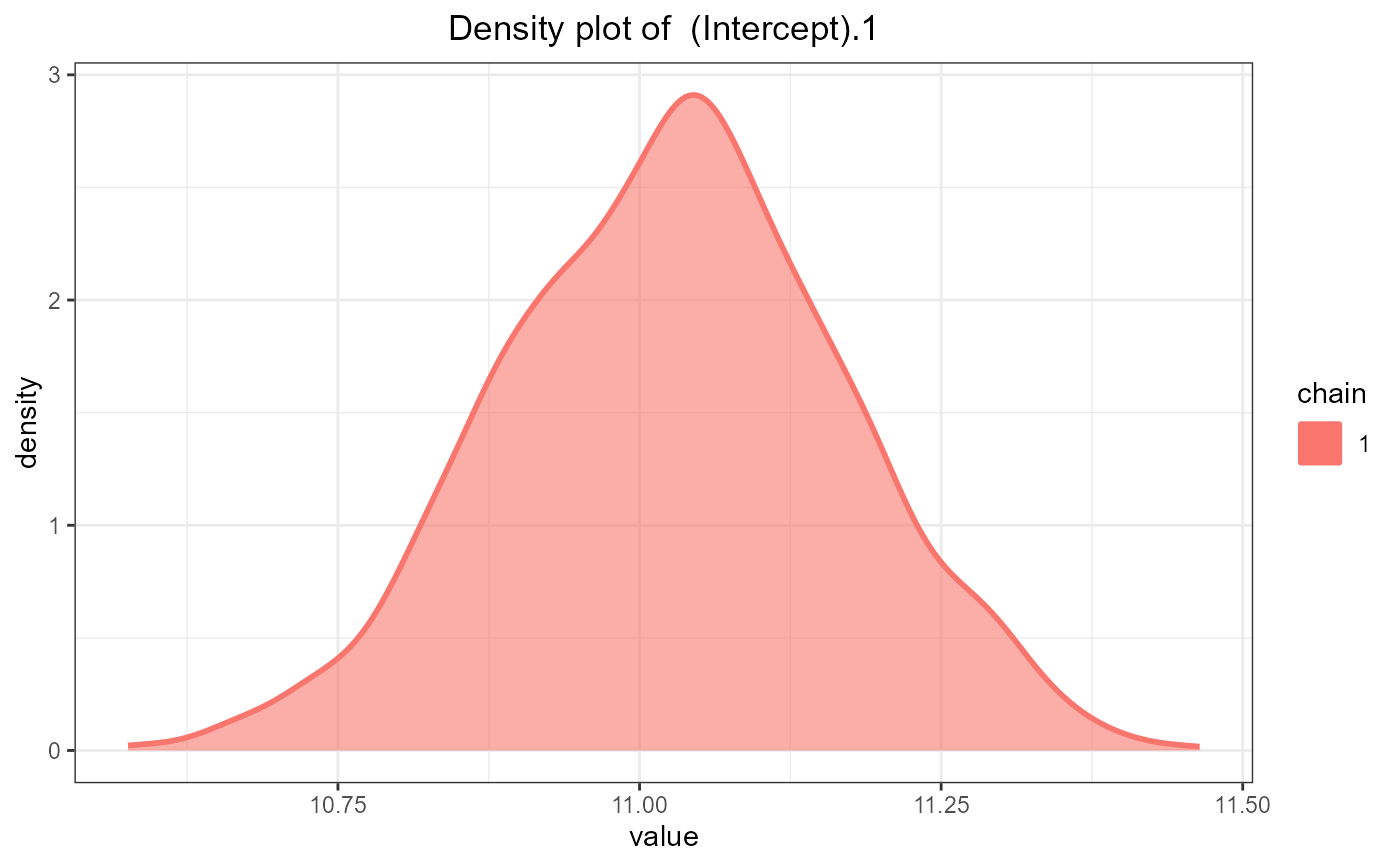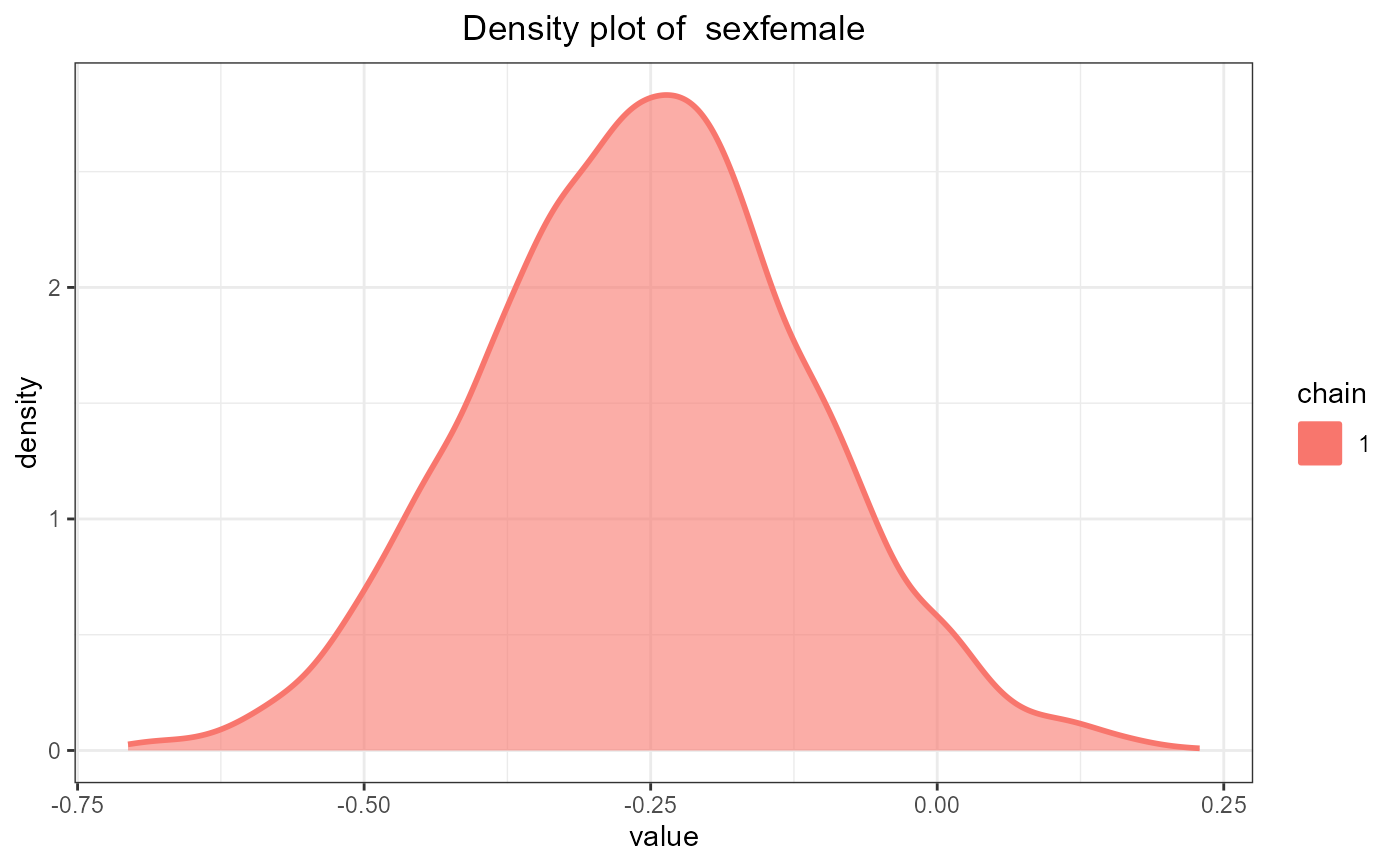ggdensityplot(fit_jm, parm = "betas", grid = TRUE)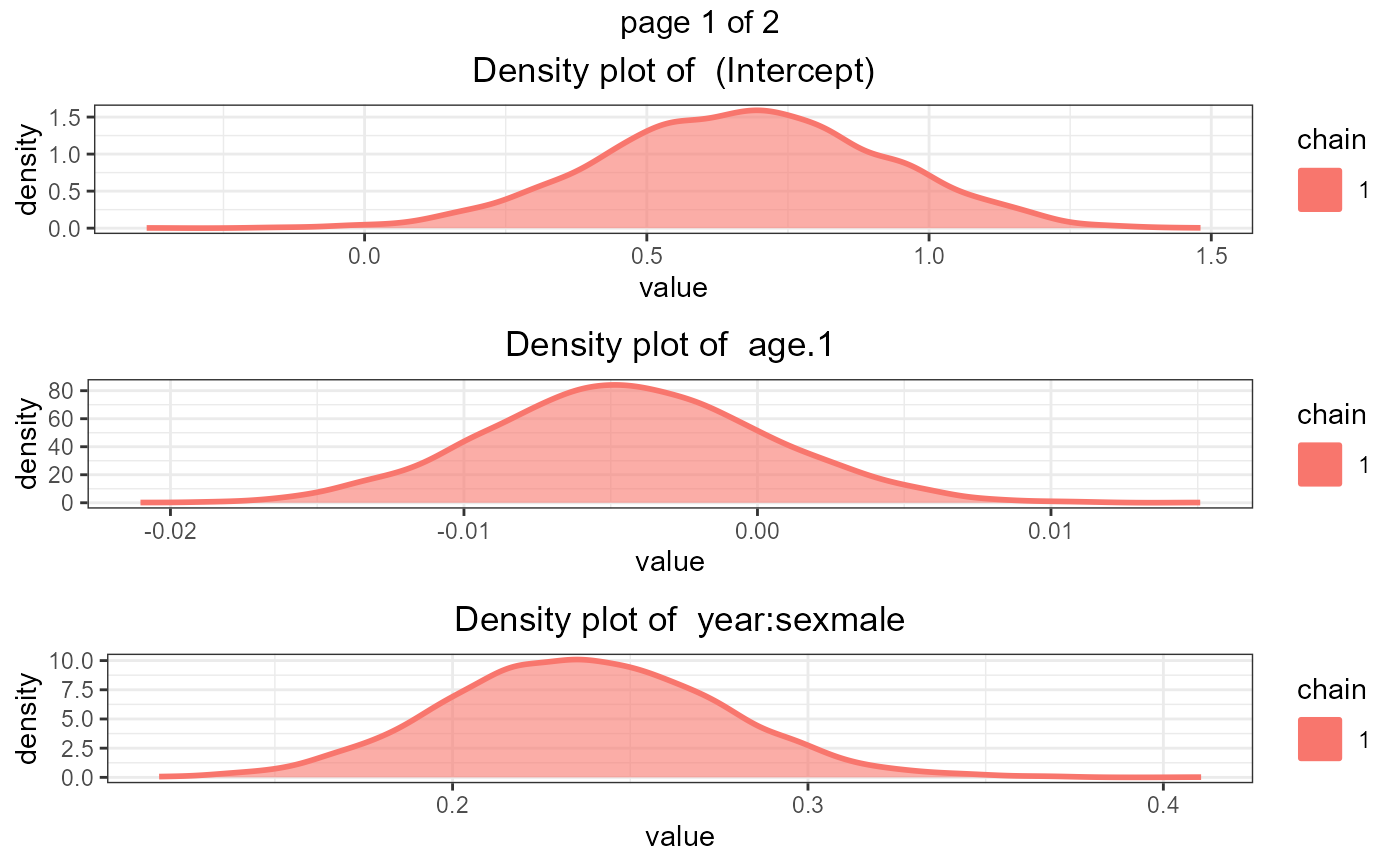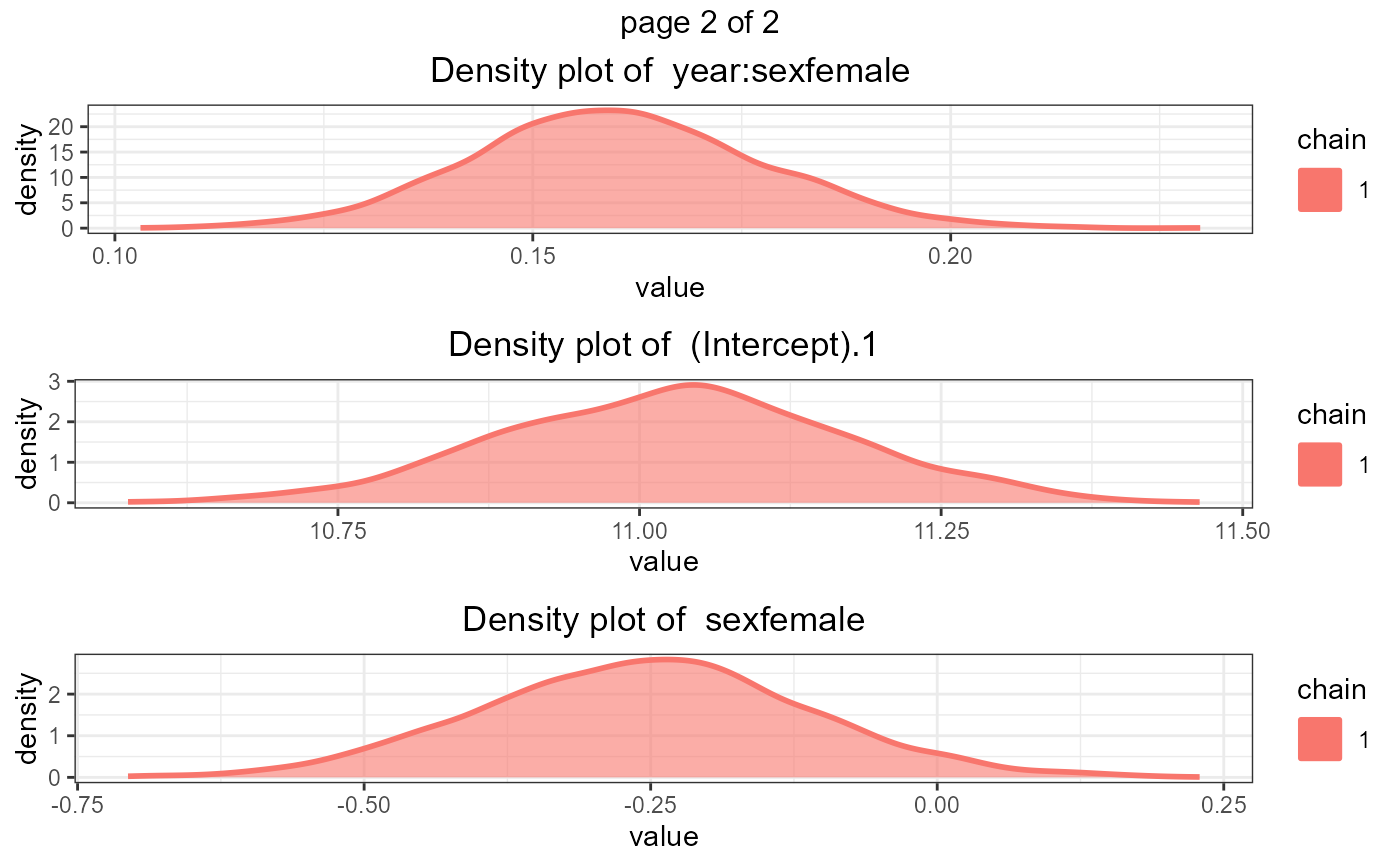ggdensityplot(fit_jm, parm = "betas", custom_theme = c('1' = 'black'))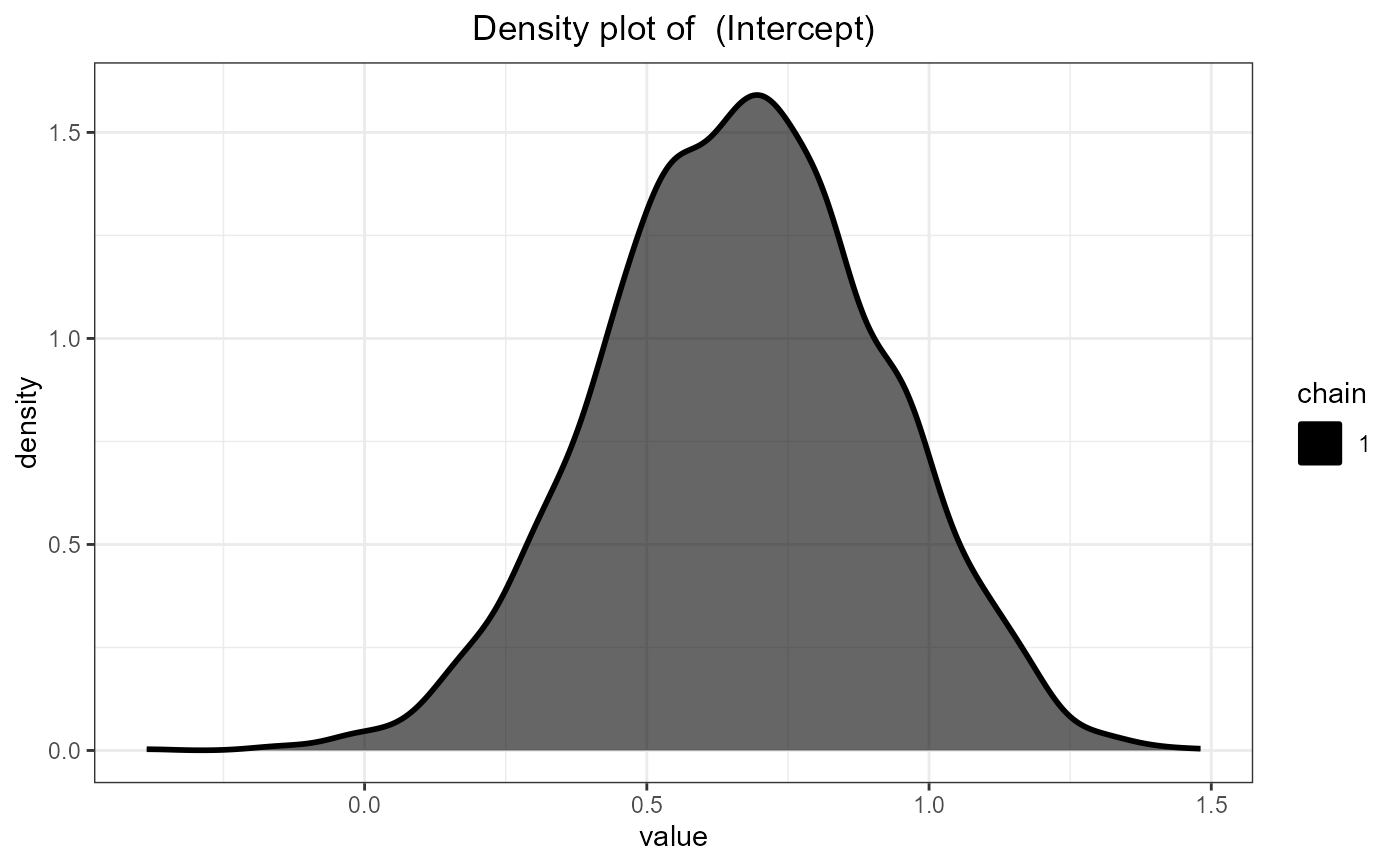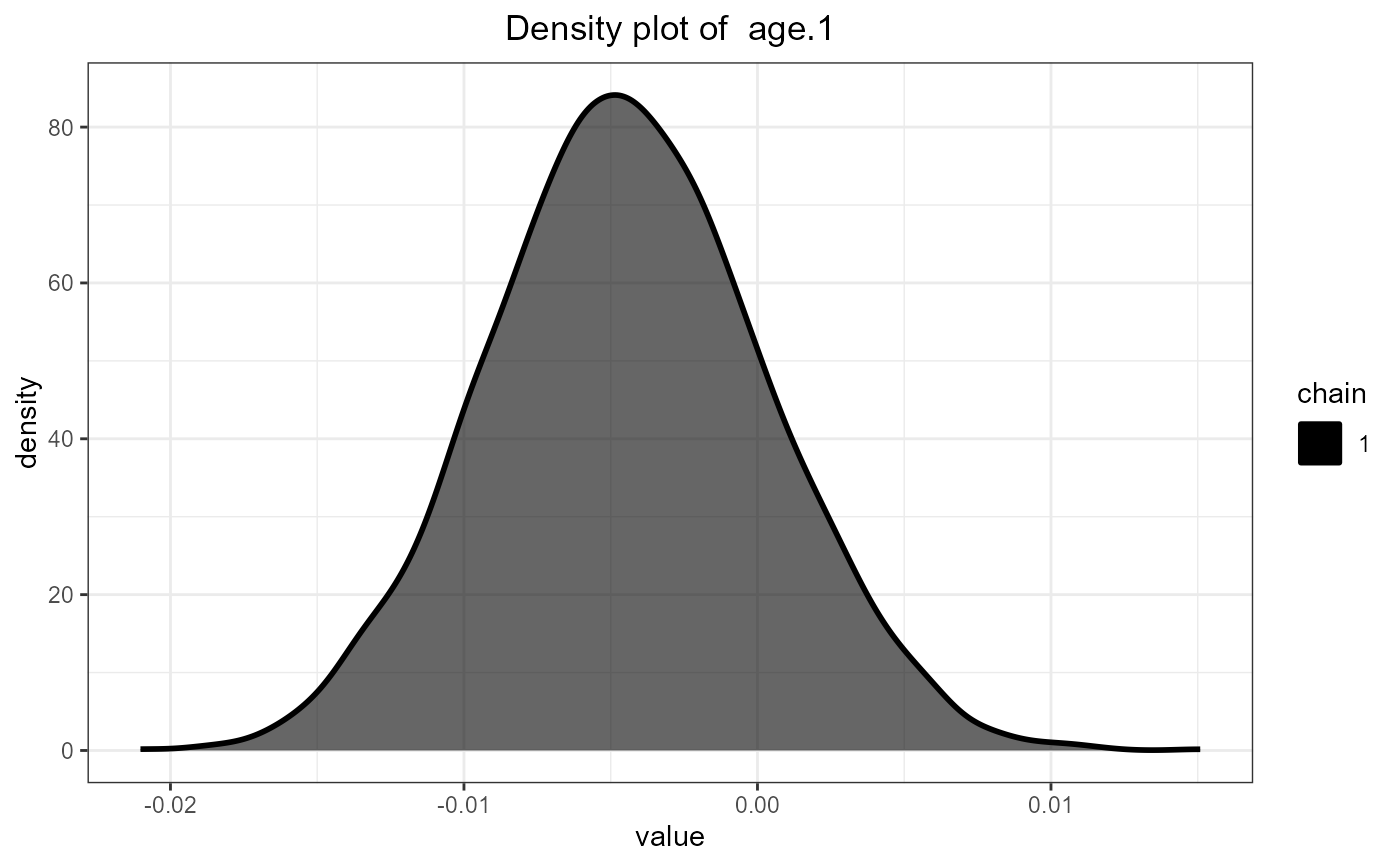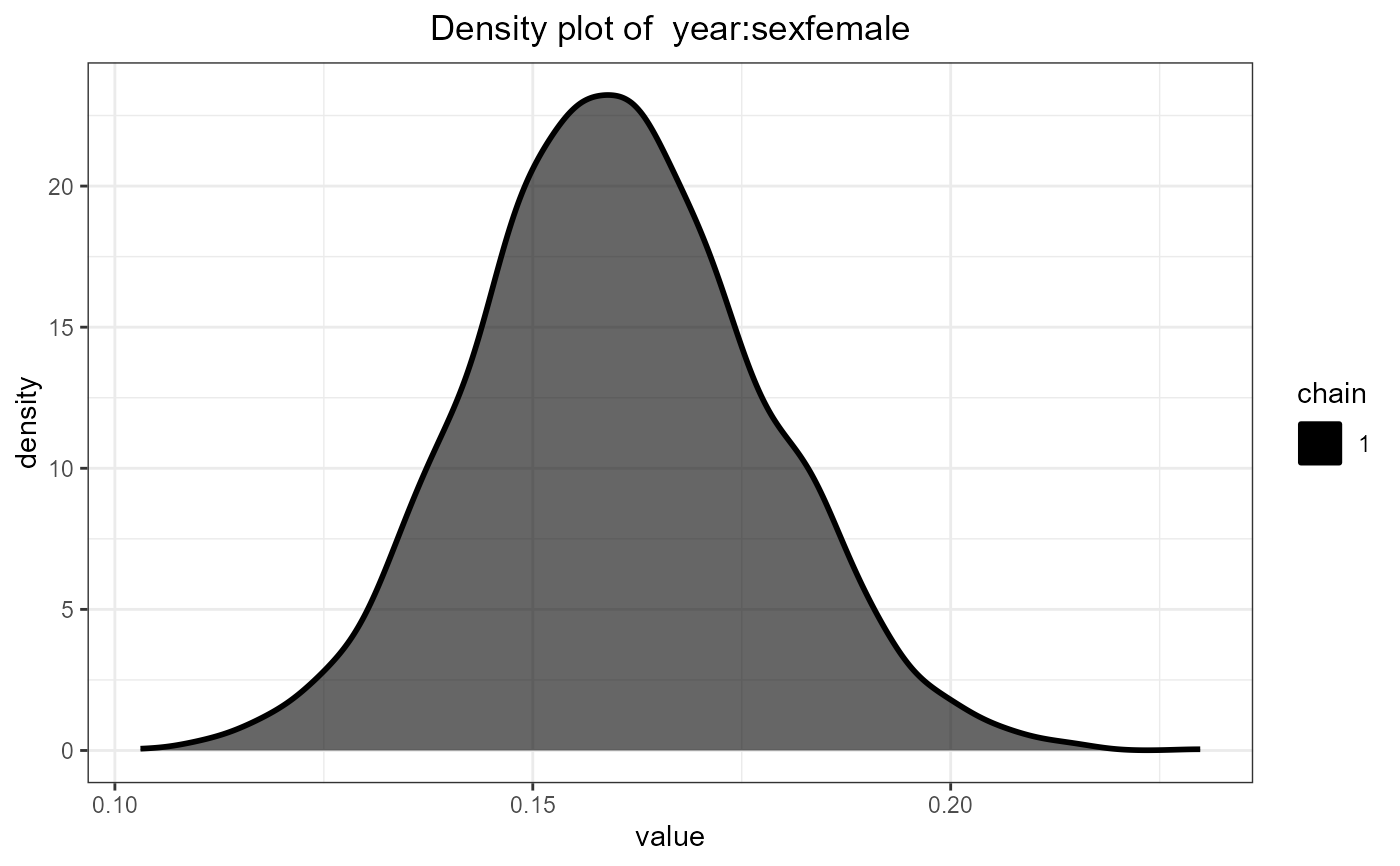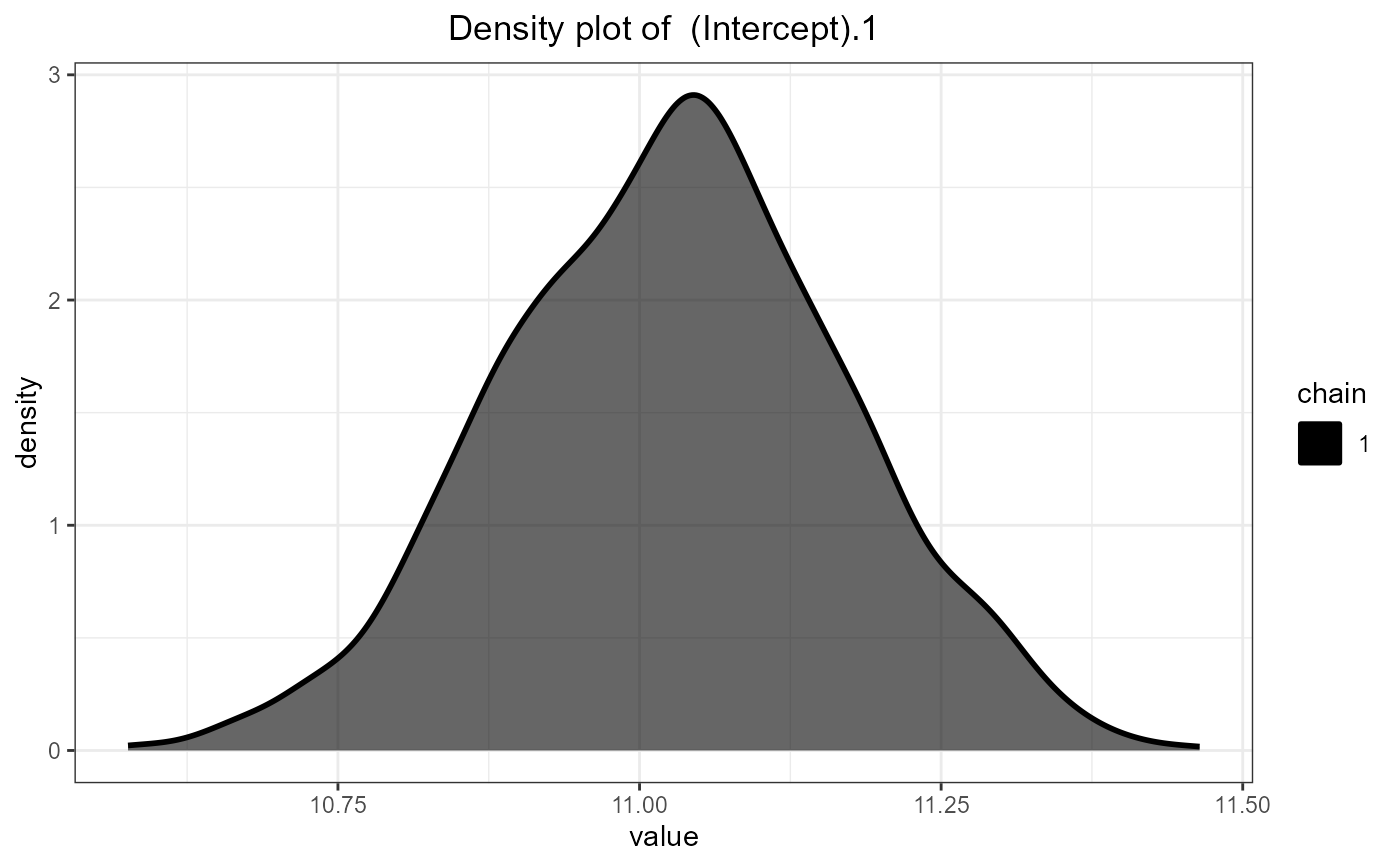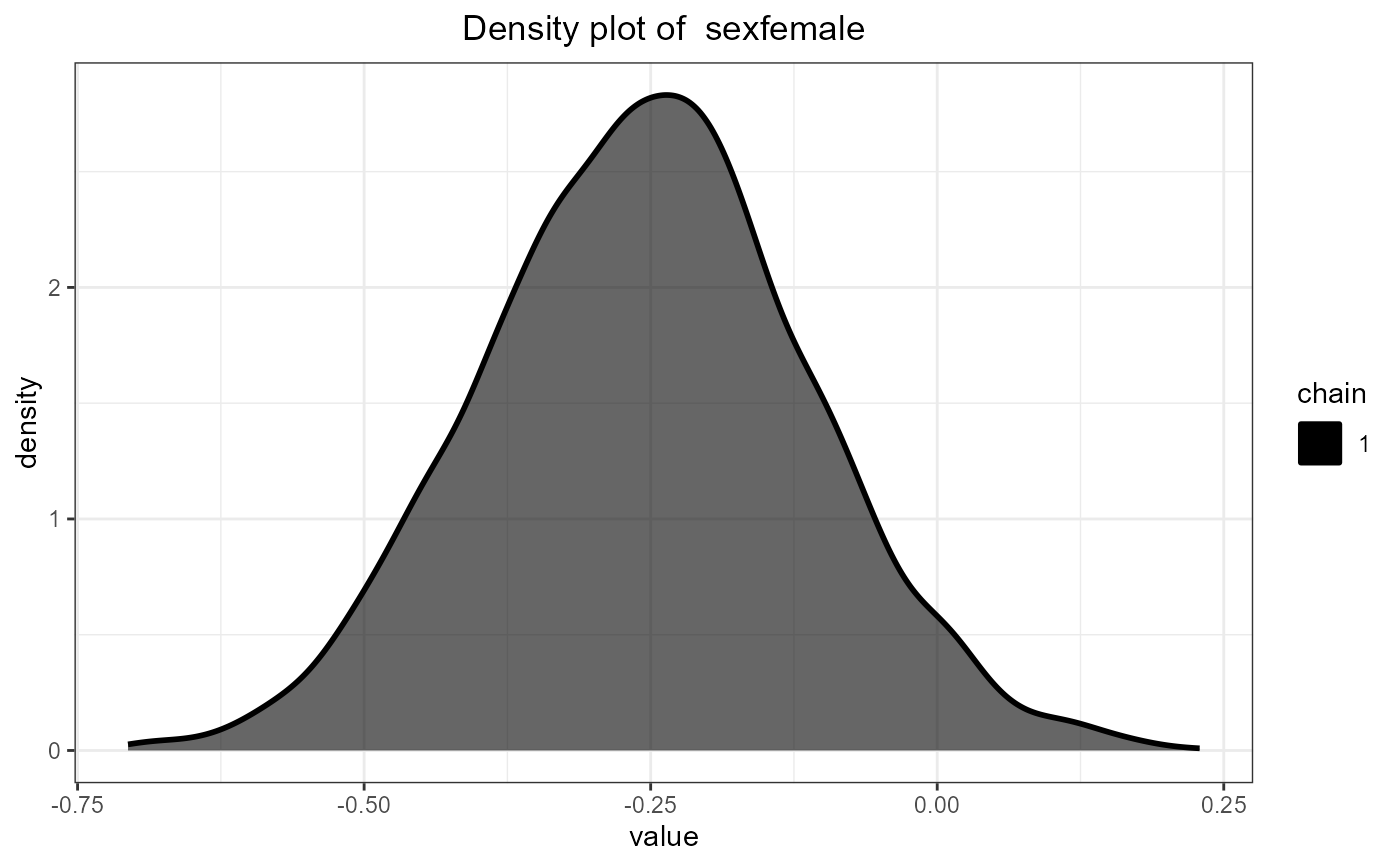# }